## Abstract

The objective of this work is the derivation and implementation of a unified Finite Element formulation for the solution of fluid and solid mechanics, Fluid-Structure Interaction (FSI) and coupled thermal problems.

The unified procedure is based on a stabilized velocity-pressure Lagrangian formulation. Each time step increment is solved using a two-step Gauss-Seidel scheme: first the linear momentum equations are solved for the velocity increments, next the continuity equation is solved for the pressure in the updated configuration.

The Particle Finite Element Method (PFEM) is used for the fluid domains, while the Finite Element Method (FEM) is employed for the solid ones. As a consequence, the domain is remeshed only in the parts occupied by the fluid.

Linear shape functions are used for both the velocity and the pressure fields. In order to deal with the incompressibility of the materials, the formulation has been stabilized using an updated version of the Finite Calculus (FIC) method. The procedure has been derived for quasi-incompressible Newtonian fluids. In this work, the FIC stabilization procedure has been extended also to the analysis of quasi-incompressible hypoelastic solids. Specific attention has been given to the study of free surface flow problems. In particular, the mass preservation feature of the PFEM-FIC stabilized procedure has been deeply studied with the help of several numerical examples. Furthermore, the conditioning of the problem has been analyzed in detail describing the effect of the bulk modulus on the numerical scheme. A strategy based on the use of a pseudo bulk modulus for improving the conditioning of the linear system is also presented.

The unified formulation has been validated by comparing its numerical results to experimental tests and other numerical solutions for fluid and solid mechanics, and FSI problems. The convergence of the scheme has been also analyzed for most of the prob- lems presented. The unified formulation has been coupled with the heat tranfer problem using a staggered scheme. A simple algorithm for simulating phase change problems is also described. The numerical solution of several FSI problems involving the temperature is given.

The thermal coupled scheme has been used successfully for the solution of an industrial problem. The objective of study was to analyze the damage of a nuclear power plant pressure vessel induced by a high viscous fluid at high temperature, the corium. The numerical study of this industrial problem has been included in this work.

## Acknowledgements

I would like to thank all the people that in some way have helped me to complete this doctoral thesis. I would like to express my gratitude to Prof. Eugenio Oñate for many reasons. Thanks to him, four years ago I could come to Barcelona and start my PhD at CIMNE. He gave me the balanced doses of freedom and pressure and he encouraged me to test innovative technologies. I would like thank Prof. Oñate also for giving me the opportunity and the material support to spend a three-month period at the College of Engineering of Swansea. Special thanks go to Dr Josep Maria Carbonell. Without his support I could not realize this work. He devoted me innumerable hours of his time and he gave me technical and not technical suggestions that helped me to make the right choices during my PhD. Moltes gràcies Josep Maria! I would like to thank Prof. Javier Bonet for giving me the possibility to work with his group during my stay at Swansea. Special thanks go to Prof. Antonio Gil y Dr. Aurelio Arranz for their very kind treatment, for improving my knowledge of the Immersed Boundary Potential Method and for the useful discussions about FSI strategies. I really hope that this is just the first step for a future fruitful collaboration. I would like to thank also Mr. Kazuto Yamamura and the Nippon Steel & Sumimoto Metal Corporation for giving us the possibility to publish the PFEM simulation of the damages of a nuclear power plant pressure vessel. I also acknowledge the Agencia de Gestió d'Ajuts Universitaris i de Recerca (AGAUR) for the financial support. I would like to thank also Riccardo, Antonia, Jordi, Pablo and Lorenzo for their contributions for this thesis. This thesis is dedicated to my parents that still represent to me a fundamental and unique support. Grazie per tutti gli sforzi che avete fatto in questi anni e per le numerose visite a Barcellona. Questa tesi è per voi. Finally, I would like to thank Lucía simply for staying by my side during these years. Her smile has been the gasoline I used for dealing with all this work.

## Abbreviations

AL Augmented Lagrangian

ASGS Algebraic SubGrid Scale

FCM Fuel Containing Material

FEM Finite Element Method

FIC Finite Increment Calculus

FSI Fluid-Structure Interaction

GLS Galerkin Least-Squares

IBM Immersed Boundary Method

IFEM Immersed Finite Element Method

ISPM Immersed Structural Potential Method

LFCM Lava-like Fuel Containing Material

NPP Nuclear Power Plant

OSS Orthogonal SubScale

PFEM Particle Finite Element Method

SPH Smooth Particle Hydrodynamics

SUPG Streamline Upwind Petrov Galerkin

TL Total Lagrangian

UL Updated Lagrangian

V Velocity

VMS Variational Multi-Scale

VP Velocity-Pressure

VPS Velocity-Pressure-Stabilized

Dai diamanti non nasce niente,
dal letame nascono i fior.

"Nothing grows out of precious diamonds,
Out of dung the flowers do grow."
Fabrizio De Andrè

# Chapter 1. Introduction

## 1.1 Objectives

The objective of this work is to develop a unified formulation for the solution of the fluid and solid mechanics, fluid-structure interaction (FSI) and thermal coupled problems. The aim is to analyze the continuum in a unified manner trying to reduce at minimum the differences between the analysis of fluids and solids. For this purpose the numerical model has been planned in order to meet the specific requirements of solid and fluid mechanics and their approximation with the Finite Element Method (FEM) , but without limiting excessively the capability of the model. In fact the computational method should be capable to deal with critical problems such as those involving elasto-plastic solids, quasi-incompressible materials, free surface fluids and phase change due to heat transfer solutions.

According to these considerations, the computational model has been designed for using a stabilized Velocity-Pressure formulation. The formulation has been particularized for hypoelasto-plastic, compressible and quasi-incompressible solids and quasi-incompressible Newtonian fluids. The algorithm for the FSI problems has been inspired by the analogous unified strategy presented in . For the fluid phase, the Particle Finite Element Method (PFEM)  has been used, while for the solid the classical FEM is adopted. The scheme has been stabilized with an updated version of the Finite Calculus (FIC) technique . For dealing with thermal coupled problems, this unified formulation has been extended to treat the heat transfer problem via a staggered scheme. For the modeling of the phase change, a simplified procedure has been developed. The whole formulation has been implemented in a C++ code.

## 1.2 State of the art

In this section, an overview of the numerical methods used for simulating a free surface fluid flow interacting with deformable solids is given. For the sake of clarity, the section is divided in three parts representing the main fields involved by this work. First the Eulerian and Lagrangian approaches for free surface fluid dynamics problems are presented. Then an overview of the stabilization for incompressible, or quasi-incompressible, material is given. Finally, the principal algorithms for solving FSI problems are described.

Consider the description of the motion of a general continuum represented in Figure 1. The domain ${\textstyle {\Omega _{0}}}$ represents the body at the initial state at time ${\textstyle {t=t_{0}}}$ while the domain ${\textstyle {\Omega }}$ represents the same body at time ${\textstyle {t=t_{n}}}$ after deformation. The domain ${\textstyle {\Omega _{0}}}$ is called initial configuration, whereas ${\textstyle {\Omega }}$ is the current, or deformed, configuration. In order to describe the kinematics and the deformation of the body, the reference configuration has to be defined because the motion is defined with respect to this configuration.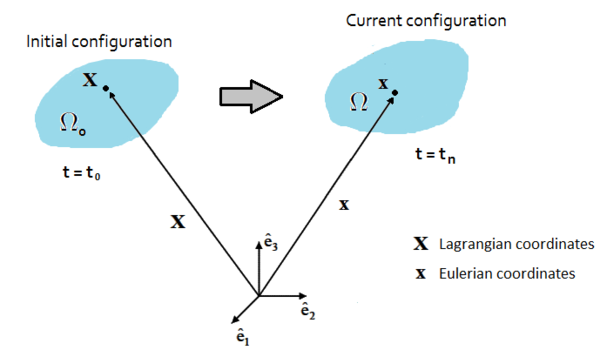Figure 1: Description of the motion of a general continuum body
In solid mechanics, the stresses generally depend on the history of deformation and the undeformed configuration must be specified in order to define the strains. Due to the history dependence, Lagrangian descriptions are prevalent in solid mechanics. In fluids however, the stresses do not depend on the history and it is often unnecessary to describe the motion with respect to a reference configuration. For this reason an Eulerian description represents the most reasonable choice. Furthermore, in problems where the fluid contours are fixed, Eulerian meshes are generally preferred to the Lagrangian ones. This is because Eulerian grids are fixed and they do not deform according to the fluid motion, as shown in Figure 2.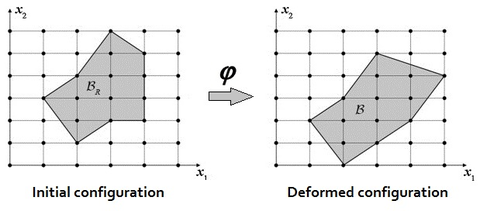Figure 2: Motion description using an Eulerian mesh
Conversely, in the Lagrangian description, the mesh nodes coincide with the fluid particles and the discretization moves and deforms as the fluid flow (Figure 3).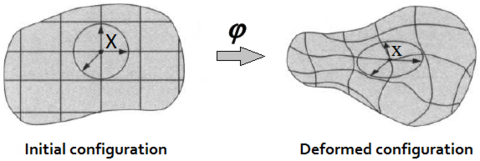Figure 3: Motion description using a Lagrangian mesh

Consequently, on the one hand the non-linear convective term disappears from the problem and on the other hand the mesh undergoes large distortions and it requires to be regenerated.

In the analysis of free surface flows, the detection of the free surface contours represents a crucial task. Its position is unknown ${\textstyle a~priori}$ and it has to be determined at each time increment in order to solve properly the boundary value problem. For these problems, the Lagrangian description may be preferred to the Eulerian one. In fact, with a Lagrangian approach the free surface is detected automatically by the position of the mesh nodes, while an Eulerian approach requires the implementation of a specific technology for this task.

Several strategies have been developed in the literature for tracking the free surface in an Eulerian framework. One of the earliest contributions was given by the so called marker and cell method . In this approach a set of marker particles that move according the flow are used to detect which regions are occupied by the fluid and which not. An evolution of this technique is the volume of fluid method . In this case the free surface boundary is detected using a scalar function that assumes the unit value in the fluid cells and the value zero in those ones with no fluid. The cells with an intermedium value are the ones that contain the free surface. Another possibility is the level set method . This technique is used in various fields, not only in continuum mechanics, and it allows for detecting shapes or surfaces on a fixed grid without making any parametrization of them. For this reason, this procedure has been also used for matching the free surface contour on an Eulerian mesh . A similar idea was used in  where the position of the free surface is detected using a cloud of Lagrangian particles moving over an Eulerian mesh.

The free surface flows can be solved also using an hybrid Eulerian-Lagrangian technique. This is the so termed Arbitrary Lagrangian-Eulerian (ALE) approach . The aim of this method is to exploit the best features of the Eulerian and the Lagrangian procedures and to combine them. The mesh nodes can arbitrary be fixed or can move with the fluid . Generally, far from the moving boundaries a fixed Eulerian grid is used, while near to the interface the mesh moves according to the motion of the boundary . However if the boundary motion is large or unpredictable, also in the ALE methods the grid may suffer large distortions and may require a proper remeshing procedure .

In purely Lagrangian approaches, the mesh needs to be regenerated whenever a threshold limit for the distortion is exceeded. This is the basis of a particular class of Lagrangian finite element formulation called the Particle Finite Element Method (PFEM). The method was initially developed by the group of professors Idelsohn and Oñate [66,67]. The PFEM treats the mesh nodes of the domain as particles which can freely move and even separate from the rest of the fluid domain representing, for instance, the effect of water drops. A mesh connects the nodes discretizing the domain where the governing equations are solved using a classical finite element method. These features make the PFEM the ideal numerical procedure to model and simulate free surface flows. In the last years, many scientific publications have shown the efficacy of the PFEM for solving free surface flow problems, see among others [32,68,117]. The PFEM has also been tested successfully in other kind of problems, such as fluid mechanics including thermal convection-diffusion [5,80,95], multi-fluids [36,62], granular materials , FSI [81,132] and excavation .

Mesh free methods are other class of Lagrangian techniques. In this strategy the remeshing is not required because the governing equations are solved over a set of nodes without referring to a mesh. One of the first meshfree techniques is the Smooth Particle Hydrodynamics (SPH) method. This method was introduced independently by Gingold and Monaghan  and Lucy  for the simulation of astrophysical problems such as fission of stars. SPH is a particle-based Lagrangian technique where discrete smoothed particles are used to compute approximate values of needed physical quantities and their spatial derivatives. The particles have assigned a characteristic distance, called ‘smoothing length’, over which their properties are ”smoothed” by a kernel function. A typical drawback of the SPH method is that it is hard to reproduce accurately the incompressibility of the materials. The SPH technique has been used successfully for solving fluid-structure interaction problems .

### 1.2.2 Stabilization techniques

A FEM-based procedure may require to be stabilized when incompressible, or quasi- incompressible, materials are analyzed. For example from the finite element solution of the Navier Stokes equations numerical instabilities may arise from two sources. The first one is due to the presence of the convective term in the linear momentum equations. This term introduces a non-linearity in the equations and it needs a proper stabilization for solving high Reynolds number flows with the FEM . Furthermore, the orders of interpolation of pressure and velocity fields cannot be chosen freely but they have to satisfy the so called ${\textstyle {Ladyzenskaya-Babuzka-Brezzi}}$ (LBB), or ${\textstyle {inf-sup}}$, condition . If the orders of interpolation of the unknown fields do not satisfy this restriction, a stabilization technique is required in order to avoid numerical instabilities, as the spurious oscillations of the pressure field.

It is well known that the weak form generated by Galerkin approximation leads to a less diffusive solution than the strong problem. So the main idea of many stabilization techniques consists of adding an artificial diffusion to the problem. The first attempt was made by Von Neumann and Richtmyer . Their solution adds an artificial diffusion to the strong form of the problem. This technique introduces an excessive dissipation to the problem because the diffusivity is added in every direction. An important evolution of this approach was the Streamline Upwind Petrov Galerkin (SUPG) . In this approach, the artificial diffusion is added by means of the test functions and only on the direction of the streamlines. Furthermore, this is performed in a consistent way: the stabilization terms vanish when the solution is reached. An extension of the SUPG method is the Galerking Least-Squares (GLS) method . The main difference between the two is that in the GLS method the stabilization terms are applied not only to the convective term but to all the terms of the equation. The Variational Multi-Scale (VMS) methods  split the problem variables in a large-scale and a subscale terms. The large-scale terms represent the part of the solution that can be captured by the finite element mesh, while the subscale part consists of an approximate solution that has to be added to the large-scale term in order to obtain the correct solution. This idea represents the basis of other stabilization methods. Among these, the most largely used are the Algebraic Subgrid Scale Formulation (ASGS) and the Orthogonal Subscales (OSS) methods, respectively introduced in [28,29]. Another efficient stabilization technique is the Finite Calculus (also termed Finite Increment Calculus) (FIC) approach (see among others [76,77,85,86]). This method has some analogies with the SUPG technique (for a comparison between these methods see ). The FIC approach is based on expressing the equations of balance of mass and momentum in a space-time domain of finite size and retaining higher order terms in the Taylor series expansion typically used for expressing the change in the transported variables within the balance domain. In addition to the standard terms of infinitesimal theory, the FIC form of the momentum and mass balance equations contains derivatives of the classical differential equations in mechanics multiplied by characteristic distances in space and time. In this work an updated version of the FIC method has been derived and tested.

In solid mechanics a stabilization procedure may be required for the solution of problems involving incompressible, or quasi-incompressible solids. Situations of this type are common in forming processes or in the analysis of the rubber-type materials. Many of the stabilization procedures for fluid dynamics have been also used also for solid dynamics. For example, the VMS method has been applied in quasi-incompressible solid mechanics in [22,24], the OSS in . In [48,49] a stabilized multi-field Petrov-Galerkin procedure is used. Finally, the application of the FIC in solid mechanics is reported [94,103].

### 1.2.3 Algorithms for FSI problems

Many approaches have been developed for solving FSI problems. Typically the computational techniques for FSI are distinguished in monolithic and staggered, or partitioned, approaches. In a monolithic approach the fluid and the solid domains are solved in a single system of equations (see among others , or ). In this technique the flow of information between the solid and the fluid parts is implicitly performed by the procedure. On the contrary, in staggered schemes the fluid and the solid dynamics are solved separately and boundary conditions are transferred from a domain to the other at the interface. From the algebraic point of view, the solution is achieved by solving two different linear systems which are coupled by means of the boundary conditions defined along the interface. Within partitioned schemes, depending on the level of coupling between the fluid and the solid dynamics, a further classification can be done. In a weakly coupled segregated schemes when the transfer of information through the interface is performed only once for each time step, (for example see ), whereas in strongly coupled schemes this operation is performed within a convergence loop (as a reference see ). Clearly, a weakly coupled scheme has a lower computational cost but it can be used only when the interaction between the solid and the fluid domains is not strong or complex. Otherwise the algorithm may not find the correct numerical solution of the problem.

Partitioned methods allow the reutilization of existing solvers. Furthermore, the solid and the fluid solvers can be updated independently. Staggered schemes lead to smaller and better conditioned linear systems than the ones obtained with monolithic approaches. On the other hand, monolithic strategies are generally more stable and fast than staggered schemes, and they lead to a more accurate solution of FSI problems, because a stronger coupling is ensured.

Another general classification of the FSI algorithms is based upon the treatment of meshes. In conforming mesh methods, in order to allow the transfer of information, the fluid and the solid meshes must have in common the nodes along the interface. Consequently, if the position of the interface nodes changes in a domain, also the other domain must modify its grid in order to guarantee the conformity of the two meshes along the interface. On the contrary, in non-conforming mesh methods the interface and the related conditions are treated as constraints imposed on the model equations so that the fluid and solid equations can be solved independently from each other with their respective grids . This represents an important advantage because, typically, the mesh used for the fluid has an average size lower than the one used for the solid and so it is not necessary to refine the solid finite element grid near to the interface. However, non-conforming mesh algorithms are more complex to implement and it is not trivial to guarantee their robustness.

In the so-called Immersed Boundary Method (${\textstyle {IBM}}$), the fluid is solved using an Eulerian grid and the solids are immersed on top of this mesh [101,104]. The interaction is ensured by penalizing the Navier-Stokes equations with the momentum forcing sources of the immersed structures. An evolution of the IBM is the ${\textstyle {Immersed~Structural}}$ ${\textstyle {Potential~Method}}$ (${\textstyle {ISPM}}$) where the structure is modeled as a potential energy functional solved over a cloud of integration points that move within the fixed fluid mesh [47,104]. Also in the ${\textstyle {Immersed~Finite}}$ ${\textstyle {Element~Method}}$ (${\textstyle {IFEM}}$) [54,129] the structure acts as a momentum forcing source for the fluid governing equations, but in this case a Lagrangian mesh is employed for the solid domains.

## 1.3 Numerical model

The aim of this work is to derive a finite element formulation capable to solve, through a unique set of equations and unknown variables, the mechanics of a general continuum. The term 'general continuum' refers to a domain that may include compressible and quasi-incompressible solids, fluids or both interacting together. For this reason, the formulation is termed ${\textstyle {Unified}}$. The Unified formulation is based on a mixed Velocity-Pressure Stabilized procedure and it has been implemented in a sequential ${\textstyle C++}$ code.

### 1.3.1 Reasons

There are many reasons for undertaking the above objective.

The first advantage of the Unified formulation is that it allows for solving fluid and solid dynamics problems by implementing and using a single calculation code.

Furthermore, if solids and fluids are solved via the same scheme, it is simpler to implement the solver for FSI problems because it is not required neither changing the variables, neither implementing the transfer of transmission conditions through the interface. With this formulation solids and fluids represent regions of the same continuum and they differ only by the specific values of the material parameters. As a consequence, the FSI solver requires a small computational effort and it can be implemented by introducing just a few specific functions. This will be explained in detail in the section dedicated to FSI problems.

Additionally, with the Unified formulation the most natural approach for solving FSI problem is the monolithic one. This brings in the further advantage that the coupling is ensured strongly and an iteration loop is not required, as for staggered procedures.

Finally, using the same set of unknowns for the fluid and the solid domains reduces the ill-conditioning of the FSI solver, because the solution system does not include variables of different units of measure.

### 1.3.2 Essential features

The Unified formulation is a compromise between a fluid and a solid formulation and it should be capable to satisfy the requirements of each problem mechanics and finite element approximations. In other words, in order to solve adequately fluids and solids with the same formulation, the solution procedure must take into account the constraints that both models impose.

Concerning the choice of the unknown variables, these are generally selected depending on the constitutive law of the materials. For Newtonian fluids, the Cauchy stress tensor is related directly to the deformation rate tensor. This implies that the velocities are the most useful unknowns in fluid mechanics. Conversely, in solid mechanics the displacements are generally preferred as variables, as the stresses are related to the deformations. However, for solids there also exist constitutive laws expressed in rate form, as for hypoelastic models.

For the analysis of incompressible, or quasi-incompressible, materials a mixed formulation is required in order to overcome the associated numerical instabilities, both in solids and fluids. In fluids, the most popular combination of unknown variables is the velocity-pressure scheme. In solid mechanics, there are several mixed approaches, as the displacement-stress , the displacement-strain , the displacement-deformation gradient , the displacement-deformation gradient-jacobian , the displacement-pressure  and the velocity-pressure formulation . The combination of unknowns is selected depending on the desired accuracy for the stress and strain fields and the associated computational cost. Among the mentioned mixed approaches, the displacement- pressure and the velocity-pressure formulations lead to the lowest computational cost, because the number of unknowns is smaller. However the velocity-pressure formula- tion has the further advantage that is the canonical scheme for fluid mechanics. Hence, according to these considerations, the Unified formulation has been based on a mixed velocity-pressure scheme.

Another key decision in the design of the Unified formulation concerns the choice of the description framework. Solids are typically solved using a (total or updated) Lagrangian description, while for fluids, due to the large deformations they undertake, the Eulerian framework is generally preferred. However, for free surface flows, as the ones treated in this work, a Lagrangian description has the important advantage that the free surface boundaries are detected automatically, because the particles of the fluid coincide with the nodes of the mesh. The price for this is that a remeshing procedure is required in order to avoid the excessive distortion of the mesh. According to these considerations, the Unified formulation has been implemented using an updated Lagrangian description.

In this work, the Particle Finite Element Method (PFEM)  has been used for solving the fluid domain only. The PFEM is a Langrangian numerical technique that treats the mesh nodes as moving points which can freely move and even separate from the domain to which they are initially attached representing, for instance, the effect of water drops. The PFEM is based on a remeshing procedure that efficiently combines the Delaunay tessellation and the Alpha Shape method . In order to reduce the computational cost associated to the remeshing step, all the unknowns are stored in the nodes and linear shape functions are used for the finite element interpolation. Conversely, the solid domain keeps a fixed mesh during the whole analysis. The reason for this is that in non-linear solid mechanics it is required to preserve not only the nodal unknowns but also elemental information as the stress state of the previous time step. A remeshing implies the elimination of the previous discretization and the creation of new elements. In order to keep the elemental information in the remeshing step, a projection procedure from the elements of the previous mesh to its nodes, and from these to the elements of the new mesh is required. These operations may introduce an interpolation error in the scheme that affects the solution of solid mechanics problems. In the analysis of Newtonian fluids all the information can be stored in the nodes, so the remeshing does not affect the accuracy of the scheme. For these reasons, in this work the PFEM is used only for the fluid, while solids are solved via the classical FEM.

In order to improve the conditioning of the solution system, a Gauss-Seidel segregated scheme is used. This means that the problem is solved iteratively and separating the unkwown variables, the velocities and the pressure. The solution method consists of two steps. In the first one the linear momentum equations are solved for the velocity increments and considering the pressure of the previous non-linear iteration in the residual term. In the second step, the continuity equation is solved for the pressure in the updated configuration using the velocities computed at the first step. This two-step algorithm leads to a smaller and better conditioned linear system than using a monolithic approach. A crucial point of this scheme is the derivation of the tangent matrix of the linear momentum equations. The velocity increments are solved by condensing the pressure. According to this procedure, the tangent matrix for the linear momentum equations of the mixed velocity-pressure formulation does not differ from the one of a velocity formulation. Furthermore, the derivation of the tangent matrix for the velocity formulation is easier, as it does not involve the pressure. For these reasons, the first step towards the unified scheme is the derivation of the Velocity formulation. The mixed Velocity-Pressure formulation will be derived by exploiting the linearization performed for the Velocity formulation. In  another procedure for the derivation of the exact tangent matrix for an Updated Lagrangian scheme is described.

The condensation of the pressure in the tangent matrix of the linear momentum equations may induce the ill-conditioning of the linear system for the analysis of quasi-incompressible materials. This ill-conditioning emanates from the volumetric counterpart of the tangent matrix that is governed by the bulk modulus, that typically has high values that may compromise the conditioning of the linear system. In this work, a thorough study of the conditioning drawbacks associated to this scheme has been performed and a useful and easy to implement technique to overcome these inconveniences has been tested and validated.

The numerical method proposed in this work is an improvement versus other numerical schemes for quasi-incompressible materials where an arbitrarily defined pseudo-bulk modulus was used in both the linear momentum and the mass conservation equations [110,109,108]. .

The method can be also related to the so-called Augmented Lagrangian (AL) procedures for solving the Navier-Stokes equations for incompressible [10,11,41,44,124] and weakly compressible [14,123].

In order to deal with incompressible (or quasi-incompressible) materials a mixed formulation is required. In this work both the velocities and the pressure are interpolated using linear shape functions. This combination does not fulfil the ${\textstyle inf-sup}$ condition  and the problem needs to be stabilized. The required stabilization is ensured using an updated version of the FIC technique  applied to the mixed Velocity-Pressure formulation. The FIC stabilization method has a small intrusivity because its terms only affect the continuity equation. In fact for solving the linear momentum equations the same scheme as for the (not stabilized) mixed Velocity-Pressure formulation is used. It will be shown that the PFEM-FIC stabilized procedure guarantees a good accuracy for the mass conservation of the free surface flows. The stabilization procedure is derived for quasi-incompressible fluids, but it can be easily extended to quasi-incompressible hypoelastic solids.

The derivation of the Unified stabilized formulation is carried on trying to maintain as much as possible the generality of the scheme. For this reason the constitutive relations are introduced only as the last step. The Velocity (V) and the mixed Velocity-Pressure (VP) formulations are derived first for a general material and then particularized for specific constitutive laws. For quasi-incompressible materials only the mixed Velocity-Pressure Stabilized (VPS) formulation can be used while for compressible materials the Velocity and the standard (not stabilized) mixed Velocity-Pressure formulations are both suitable. In particular, for compressible solids, the hypoelastic model has been chosen.

The FSI problem is solved in a monolithic way, hence solids and fluids are solved by the same linear system. For the solid domain it is possible to choose which formulation to use. Depending on the problem, one may chose the V, the VP or the VPS element.

The Unified formulation for FSI problems can be easily coupled with the heat transfer problem in order to solve coupled thermal mechanical problem. The coupling is performed via a staggered scheme. The effect of the heat is taken into account by considering the material properties depending on the temperature and including in the strain tensor the deformations induced by the temperature. With the PFEM Unified formulation also phase change problems can be modeled.

The Unified stabilized VP formulation with thermal coupling has been used for the analysis of an industrial application. The study concerned the analysis of the damages caused by the dropping of a volume of corium at high temperature on the structure of the pressure vessel in a Nuclear Power Plant (NPP).

### 1.3.3 Outline

This text is split in the following chapters.

In the next chapter two velocity-based FEMs are derived for a general compressible material. In the first section the Velocity formulation is derived and the incremental solution scheme is given. Then, the standard mixed Velocity-Pressure formulation is obtained as an extension of the Velocity formulation. The constitutive laws are not introduced for any scheme. In the third section the formulations are adapted for hypoelastic-plastic compressible solids. The chapter ends with several validation examples for non-linear solid mechanics.

In Chapter 3, the FIC stabilization strategy is introduced in the mixed stabilized VP scheme. This scheme is adapted for quasi-incompressible Newtonian fluids and hypoelastic solids, in this order. Then the free surface problem is studied in detail. First the PFEM is described highlighting the advantages and the disadvantages of the method. A useful technique for modeling the slip conditions in Lagrangian flows is also explained. Next the mass conservation feature and the conditioning of the scheme are analyzed in detail. Concerning the former point, it will be shown that the PFEM-FIC formulation guarantees a good preservation of the mass in free surface flows. Regarding the latter point, a practical strategy for improving the conditioning of the linear system is explained and tested. At the end of the chapter several validation examples for quasi-incompressible Newtoninan fluids and hypoelastic solids are given.

The FSI solution strategy is described in Chapter 4. The monolithic scheme for coupling the mechanics of fluids and solids is explained in detail. Finally several validation examples of FSI problems are given.

Chapter 5 is dedicated to couple the Unified formulation for FSI with the heat problem. In the first section the heat problem is introduced and discretized using the FEM. Then the coupling strategy is explained and validated with numerical examples. Next, the procedure for modeling phase change problems is described and an explicative numerical example is presented.

Chapter 6 is fully devoted to the industrial application of the Unified stabilized and thermally coupled strategy. The damages of the pressure vessel structure caused by the fall of a volume corium at high temperature are studied using two simplified models where the solid structure melts due to the heat transfer from the corium.

In Chapter 7 the innovative contributions of the work are summarized and the lines of research opened by this thesis are presented.

## 1.4 Publications

The following publications have emanated from the work carried out in this doctoral thesis

1. E. Oñate and A. Franci and J.M. Carbonell, Lagrangian formulation for ﬁnite ele-ment analysis of quasi-incompressible ﬂuids with reduced mass losses, International Journal for Numerical Methods in Fluids, 74 (10), 699-731, 2014;
2. E. Oñate and A. Franci and J.M. Carbonell, A particle ﬁnite element method (PFEM) for coupled thermal analysis of quasi and fully incompressible ﬂows and ﬂuid-structure interaction problems, Numerical Simulations of Coupled Problems in Engineering. S.R. Idelsohn (Ed.), 33, 129-164, 2014;
3. E. Oñate and A. Franci and J.M. Carbonell, A particle ﬁnite element method for analysis of industrial forming processes, Computational Mechanics, DOI: 10.1007/s00466-014-1016-2, 2014;
4. A. Franci and E. Oñate and J.M. Carbonell, On the eﬀect of the bulk tangent ma-trix in partitioned solution schemes for nearly incompressible ﬂuids, International Journal for Numerical Methods in Engineering, DOI: 10.1002/nme.4839, 2014;
5. A. Franci and E. Oñate and J.M. Carbonell, Uniﬁed formulation for solid and ﬂuid mechanics and FSI problems, Computer Methods in Applied Mechanics and Engineering, (in preparation).

# Chapter 2. Velocity-based formulations for compressible materials

In this chapter two velocity-based finite element formulations for compressible materials are presented, namely the Velocity (V) and the mixed Velocity-Pressure (VP) formulations. For both schemes the linear momentum equations are solved iteratively for the velocity increments. The linearization of the governing equations is performed without specifying any constitutive law. The aim of this chapter is to maintain as much as possible the generality of the algorithms, leaving the formulations open to different material models. It will be shown that the only requirement demanded to the constitutive laws is that the rate of stress must be linearly related with the rate of deformation.

There are several reasons that justify the presentation of the Velocity formulation. First of all, the tangent matrix of the linear momentum equations for the Velocity formulation holds also for the mixed Velocity-Pressure formulation but the linearization procedure is easier because the pressure does not appear. Furthermore, the Velocity formulation is useful for making some interesting and didactic comparisons with the mixed formulations.

After deriving the solution scheme for the Velocity formulation, the mixed Velocity-Pressure method is presented. The governing equations are the linear momentum and the linear pressure-deformation rate equations. The latter is called continuity, or mass balance, equation for its similarity with the incompressibility constraint of the Navier-Stokes problem. As for the velocity scheme, in the mixed formulation the constitutive law is not specified. In Section 2.3 the hypoelastic-plastic model is presented and this constitutive law is inserted in both the Velocity and the mixed Velocity-Pressure schemes. The incremental solution scheme is explained in detail for both formulations. At the end of the chapter some validation examples for hypoelastic-plastic solids in statics as in dynamics are given.

## 2.1 Velocity formulation

In this section, the velocity formulation for solving transient problems for a general continuum is derived. The governing equations are the linear momentum equations and they are derived in the updated Lagrangian (UL) framework. This means that the governing equations are integrated over the unknown configuration ${\textstyle {\Omega }}$ (the so-called updated configuration). As a consequence, the space derivatives for the UL description are computed with respect to the spatial coordinates.

### 2.1.1 From the local form to the spatial semi-discretization

In this section the spatial semi-discretization of the linear momentum equations is derived.

For a general continuum, the local form of the linear momentum equations using the UL description reads

 $\rho ({\hbox{X}},t){\frac {\partial v({\hbox{X}},t)}{\partial t}}-{\partial \sigma ({\hbox{X}},t) \over \partial {\hbox{x}}}-b({\hbox{X}},t)=0\quad \quad in\Omega \times (0,T)$
(1)

where ${\textstyle \rho }$ is the density of the material, ${\textstyle v}$ are the velocity vector, ${\textstyle \sigma }$ is the Cauchy stress tensor and ${\textstyle b}$ is the body force vector. The variables within the brackets are the independent variables: X are the Lagrangian or material coordinates vector, x the Eulerian or spatial coordinates vector and ${\textstyle t}$ is the time. For simplicity, in what follows the independent variables are not specified. The spatial and material coordinates are related through the motion tensor ${\textstyle {\Phi }}$ as

 ${\hbox{x}}=\Phi ({\hbox{X}},t),{\hbox{X}}=\Phi ^{-1}({\hbox{x}},t)$
(2)

The set of governing equations is completed by the following conditions at the Dirichlet (${\textstyle \Gamma _{v}}$) and Neumann (${\textstyle \Gamma _{t}}$) boundaries

 $v_{i}-v_{i}^{p}=0\qquad {\hbox{on }}\Gamma _{v}$
(3)

 $\sigma _{ij}n_{j}-t_{i}^{p}=0\qquad {\hbox{on }}\Gamma _{t}$
(4)

where ${\textstyle v_{i}^{p}}$ and ${\textstyle t_{i}^{p}}$ are the prescribed velocities and the prescribed tractions, respectively, and ${\textstyle n}$ is the unit normal vector.

In the following summation of terms for repeated indices is assumed, unless otherwise specified.

The spaces for the trial and test functions are defined, respectively, as

 $v_{i}\in {U},\quad \quad {U}=\{v_{i}|v_{i}\in C^{0},v_{i}=v_{i}^{p}on\Gamma _{v}\}$
(5)

 $w_{i}\in {U_{0}},\quad \quad {U_{0}}=\{w_{i}|w_{i}\in C^{0},w_{i}=0on\Gamma _{v}\}$
(6)

Multiplying Eqs.(1) by the test functions and integrating over the updated configuration domain, the following global integral form is obtained

 $\int _{\Omega }w_{i}\left(\rho {\dot {v}}_{i}-{\partial \sigma _{ij} \over \partial x_{j}}-b_{i}\right)d\Omega =0$
(7)

where the symbol ${\textstyle {\dot {(\cdot )}}}$ represents the material time derivative.

Integrating by parts the term involving ${\textstyle \sigma _{ij}}$ in Eq.(7) and using the Neumann boundary conditions (4) yields the weak variational form of the momentum equations as

 $\int _{\Omega }w_{i}\rho {\dot {v}}_{i}d\Omega +\int _{\Omega }{\frac {\partial w_{i}}{\partial x_{j}}}\sigma _{ij}d\Omega -\int _{\Omega }w_{i}b_{i}d\Omega -\int _{\Gamma _{t}}w_{i}t_{i}^{p}d\Gamma =0$
(8)

Eq.(8) is the standard form of the Principle of Virtual Power .

The spatial discretization is introduced using the classical FEM-Galerkin procedure . Hence both the trial and the test functions are interpolated in space in terms of their nodal values by means of the same shape functions ${\textstyle {N}}$

 $v_{i}=\sum \limits _{I=1}^{n}N_{I}(X){\bar {v}}_{iI}\quad ,\quad w_{i}=\sum \limits _{I=1}^{n}N_{I}(X){\bar {w}}_{iI}$
(9)

where, assuming the use of simplicial elements, ${\textstyle n=3/4}$ for 2D/3D problems is the number of the nodes of the element, ${\textstyle {\bar {(\cdot )}}}$ denotes a nodal value, the capital subscript specifies the node and the lower case subscript represents the cartesian direction. In this work, linear shape functions have been used for ${\textstyle N_{I}}$.

Since Eq.(9) must hold for all the test functions in the interpolation space, introducing the spatial discretization (9) into Eq.(8), the spatial semi-discretized form of the momentum equations in the UL framework for the node ${\textstyle {I}}$ reads

 $\underbrace {\int _{\Omega }N_{I}\rho d\Omega {\dot {v_{i}}}} _{{f}_{Ii}^{dyn}}}+\underbrace {\int _{\Omega }{\frac {\partial N_{I}}{\partial x_{j}}}\sigma _{ij}d\Omega } _{{f}_{Ii}^{int}}}=\underbrace {\int _{\Omega }N_{I}b_{i}d\Omega +\int _{\Gamma _{t}}N_{I}t_{i}^{p}d\Gamma } _{{f}_{Ii}^{ext}}}$
(10)

where ${\textstyle {f^{dyn}}}$, ${\textstyle {f^{int}}}$ and ${\textstyle {f^{ext}}}$ are the dynamic, internal and external forces, respectively, expressed in the UL framework.

For convenience, the semi-discretized form of the momentum equations in the total Lagrangian (TL) framework is also presented here. This is written as 

 $\underbrace {\int _{\Omega _{0}}N_{I}\rho _{0}d\Omega {\dot {v_{i}}}} _{^{TL}{f}_{Ii}^{dyn}}}+\underbrace {\int _{\Omega _{0}}{\frac {\partial N_{I}}{\partial X_{j}}}P_{ij}d\Omega } _{^{TL}{f}_{Ii}^{int}}}=\underbrace {\int _{\Omega _{0}}N_{I}b_{i}d\Omega +\int _{\Gamma _{0}}N_{I}t_{0i}^{p}d\Gamma } _{^{TL}{f}_{Ii}^{ext}}}$
(11)

where ${\textstyle {P}}$ is the first Piola-Kirchhoff stress tensor, or the nominal stress tensor, and ${\textstyle {^{TL}f^{dyn}}}$, ${\textstyle {^{TL}f^{int}}}$ and ${\textstyle {^{TL}f^{ext}}}$ are the dynamic, internal and external forces, respectively, expressed in the TL framework. All the variables with vectors subscript ${\textstyle {(\cdot )}_{0}}$ refer to the last known configuration. Note that Eq.(11) can be obtained from Eq.(10) by pull back transformations on all its terms .

For the sake of clarity in the notation, the terms referred to the TL description are denoted with the left index ${\textstyle {^{TL}(\cdot )}}$. Unless otherwise specified, the variables belong to the UL description.

### 2.1.2 Time integration

In this work, the kinematic variables have been integrated in time using a second order scheme. In particular, the implicit Newmark's integration rule has been adopted. For the general case, this rule states that accelerations and displacements are computed as

 $^{n+1}{\dot {v}}$ $={\frac {1}{\gamma \Delta t}}\left({^{n+1}v}-{^{n}v}\right)-{\frac {1-\gamma }{\gamma }}{^{n}{\dot {v}}}$ $^{n+1}u$ $={^{n}u}+{\Delta t}{\frac {\gamma -\beta }{\gamma }}{^{n}v}+\Delta t{\frac {\beta }{\gamma }}{^{n+1}v}+{\Delta t^{2}}{\frac {\gamma {-}2\beta }{2\gamma }}{^{n}{\dot {v}}}$
(12)

Where ${\textstyle {\beta }}$ and ${\textstyle {\gamma }}$ are the so-called Newmark's parameters . This time integration scheme is unconditionally stable if the following relation holds

 $\gamma \geq 2\beta \geq {\frac {1}{2}}$
(13)

In the present work, the Newmark's parameters chosen are ${\textstyle {\beta ={\frac {1}{4}}}}$ and ${\textstyle {\gamma ={\frac {1}{2}}}}$.

Replacing the numerical values of the constants in Eq.(12) yields

 $^{n+1}{\dot {v}}={\frac {2}{\Delta t}}\left({^{n+1}v}-{^{n}v}\right)-{^{n}{\dot {v}}}$
(14)

 $^{n+1}u={^{n}u}+{\frac {\Delta t}{2}}\left({^{n+1}v}+{^{n}v}\right)$
(15)

### 2.1.3 Linearization

Although the problem is set out in the UL framework, the linearization for the velocities increment of the momentum equations is performed first on the TL semi-discretized form (11). The UL linearized form is obtained by performing a push-forward transformation on the TL form. This is justified by the easier derivation of the tangent matrix in the TL framework. In fact, in Eq.(11) the only variable that depends on time is the nominal stress ${\textstyle {P}}$, while in the UL form (10) the time-dependent variables are the updated domain ${\textstyle {\Omega }}$, the Cauchy stress tensor ${\textstyle {\sigma }}$ and the spatial derivatives ${\textstyle {\partial N/\partial x}}$. For the sake of clarity, the linearization of the internal and dynamic forces will be performed separately.

Internal component of the tangent matrix

From Eq.(11) the internal forces in the TL description are defined as

 $^{TL}f_{Ii}^{int}=\int _{\Omega _{0}}{\frac {\partial N_{I}}{\partial X_{j}}}P_{ij}d\Omega _{0}$
(16)

The constitutive relation is expressed in rate form. Hence it is more convenient to perform the linearization of the material derivative of the internal forces and then integrate for the time step increment ${\textstyle \Delta t}$. The material time derivative of (16) is

 $^{TL}{\dot {f}}_{Ii}^{int}=\int _{\Omega _{0}}{\frac {\partial N_{I}}{\partial X_{j}}}{\dot {P}}_{ij}d\Omega _{0}$
(17)

The infinitesimal increment of Eq.(17) is

 $^{TL}\delta {\dot {f}}_{Ii}^{int}=\int _{\Omega _{0}}{\frac {\partial N_{I}}{\partial X_{j}}}\delta {\dot {P}}_{ij}d\Omega _{0}$
(18)

The first Piola-Kirchhoff stress tensor ${\textstyle {P}}$ is not typically used because it is not symmetric and its rate is a non-objective measure. For these reasons, in the TL framework it is more convenient to work with the second Piola-Kirchhoff stress tensor ${\textstyle {S}}$ and its rate. These stress rate measures are related each other through the following relation

 ${\dot {P}}_{ij}={\dot {S}}_{ir}F_{rj}^{T}+S_{ir}{\dot {F}}_{rj}^{T}$
(19)

where ${\textstyle {F}}$ is the deformation gradient tensor defined as

 ${F}_{ij}={\frac {\partial x_{i}}{\partial X_{j}}}$
(20)

Substituting Eq.(19) into (18) yields

 $^{TL}\delta {\dot {f}}_{Ii}^{int}=\underbrace {\int _{\Omega _{0}}{\frac {\partial N_{I}}{\partial X_{j}}}F_{ir}\delta {\dot {S}}_{jr}d\Omega _{0}} _{^{TL}\delta {\dot {f}}_{Ii}^{m}}}+\underbrace {\int _{\Omega _{0}}{\frac {\partial N_{I}}{\partial X_{j}}}S_{ir}\delta {\dot {F}}_{rj}^{T}d\Omega _{0}} _{^{TL}\delta {\dot {f}}_{Ii}^{g}}}$
(21)

Eq.(21) shows that the increment of the material time derivative of the internal forces can be split into material and geometric parts, ${\textstyle {^{TL}\delta {\dot {f}}^{m}}}$ and ${\textstyle {^{TL}\delta {\dot {f}}^{g}}}$, respectively. The former accounts for the material response through the rate of the second Piola-Kirchhoff stress tensor and the second term is the initial stress term that contains the information of the updated stress field. Note that, up to now, no constitutive relationships have been introduced and the above derivation holds for a general continuum.

Material tangent matrix

From Eq.(21), the material part of the material time derivative of the internal forces reads

 ${^{TL}\delta {\dot {f}}_{Ii}^{m}=\int _{\Omega _{0}}{\frac {\partial N_{I}}{\partial X_{j}}}F_{ir}\delta {\dot {S}}_{jr}d\Omega _{0}}$
(22)

For the derivation of the material tangent matrix, the constitutive relation between the stress and the strain measures is required. In order to maintain the formulation as general as possible, the stress rate measure is related to the deformation rate through a tangent constitutive tensor, as

 ${\dot {S}}_{ij}=C_{ijkl}{\dot {E}}_{kl}$
(23)

where ${\textstyle {C}}$ is a fourth-order tensor and ${\textstyle {E}}$ is the Green-Lagrange strain tensor. Eq.(23) can also be expressed in Voigt notation as

 $\{{\dot {S}}\}=\left[C\right]\{{\dot {E}}\}$
(24)

where ${\textstyle {(\cdot )}}$ denotes a vector with components ${\textstyle \left[(\cdot )_{11},(\cdot )_{22},(\cdot )_{33},(\cdot )_{12},(\cdot )_{13},(\cdot )_{23}\right]}$. As it will be shown in the following sections, Eq.(23) can represent both a Kirchhoff solid material and a Newtonian fluid. If different constitutive laws are used, Eq.(23) should be modified accordingly in order to derive the material part of the tangent matrix.

The Green-Lagrange strain tensor can be expressed in terms of the nodal velocities as

 ${\dot {E}}_{kl}={\frac {\partial N_{J}}{\partial X_{l}}}F_{sk}{\bar {v}}_{Js}$
(25)

In Voigt notation

 $\{{\dot {E}}\}=B_{0}{\bar {v}}$
(26)

where for a plane strain problem

 $\displaystyle {B_{0}}=\left[{\begin{matrix}\displaystyle {\frac {\partial N_{I}}{\partial {X}}}{\frac {\partial x}{\partial {X}}}&\displaystyle {\frac {\partial N_{I}}{\partial {X}}}{\frac {\partial y}{\partial {X}}}\\[.4cm]\displaystyle {\frac {\partial N_{I}}{\partial {Y}}}{\frac {\partial x}{\partial {Y}}}&\displaystyle {\frac {\partial N_{I}}{\partial {Y}}}{\frac {\partial y}{\partial {Y}}}\\[.4cm]\displaystyle {\frac {\partial N_{I}}{\partial {X}}}{\frac {\partial x}{\partial {Y}}}+{\frac {\partial N_{I}}{\partial {Y}}}{\frac {\partial x}{\partial {X}}}&\displaystyle {\frac {\partial N_{I}}{\partial {X}}}{\frac {\partial y}{\partial {Y}}}+{\frac {\partial N_{I}}{\partial {Y}}}{\frac {\partial y}{\partial {X}}}\\\end{matrix}}\right]$
(30)

Substituting Eq.(25) in Eq.(23), yields

 ${\dot {S}}_{ij}=C_{ijkl}{\frac {\partial N_{J}}{\partial X_{l}}}F_{sk}{\bar {v}}_{Js}$
(31)

Similarly, substituting Eq.(26) in Eq.(24)

 $\{{\dot {S}}\}=\left[C\right]B_{0}v$
(32)

Substituting Eq.(31) into Eq.(22) yields

 ${^{TL}\delta {\dot {f}}_{Ii}^{m}}=\int _{\Omega _{0}}{\frac {\partial N_{I}}{\partial X_{j}}}F_{ir}C_{jrkl}{\frac {\partial N_{J}}{\partial X_{l}}}F_{sk}d\Omega _{0}\delta {\bar {v}}_{Js}$
(33)

where ${\textstyle {{\bar {v}}_{Js}}}$ is the ${\textstyle {s}}$-component of the velocity of node ${\textstyle {J}}$. In Voigt notation Eq.(33) reads

 ${^{TL}\delta {\dot {f}}^{m}}=\int _{\Omega _{0}}B_{0}^{T}\left[C\right]B_{0}d\Omega _{0}\delta {\bar {v}}$
(34)

In order to obtain the increment of the internal forces, the material time derivative of the internal forces increment is integrated over a time step increment ${\textstyle \Delta t}$ as

 ${^{TL}\delta {f}^{m}}={^{TL}\delta {\dot {f}}^{m}}\Delta t$
(35)

From Eq.(35) and Eq.(33), yields

 ${^{TL}\delta {f}_{Ii}^{m}}=\int _{\Omega _{0}}{\frac {\partial N_{I}}{\partial X_{j}}}F_{ir}\Delta tC_{jrkl}{\frac {\partial N_{J}}{\partial X_{l}}}F_{sk}d\Omega _{0}\delta {\bar {v}}_{Js}$
(36)

and, similarly, from Eq.(35) and Eq.(34)

 ${^{TL}\delta {f}^{m}}=\int _{\Omega _{0}}B_{0}^{T}\Delta t\left[C\right]B_{0}d\Omega _{0}\delta {\bar {v}}$
(37)

From Eq.(36) and Eq.(37), the material tangent matrix in the TL description can be computed as

 $^{TL}{K}_{IJis}^{m}=\int _{\Omega _{0}}{\frac {\partial N_{I}}{\partial X_{j}}}F_{rj}\Delta tC_{ijkl}{\frac {\partial N_{J}}{\partial X_{s}}}F_{kl}d\Omega$
(38)

 ${^{TL}{K}^{m}}=\int _{\Omega _{0}}B_{0}^{T}\Delta t\left[C\right]B_{0}d\Omega _{0}$
(39)

The material tangent matrix for the UL framework is obtained by applying a push-forward transformation on each term of Eq.(38) and integrating over the updated domain ${\textstyle \Omega }$. The following relations hold

 $d{\Omega _{0}}={\frac {d\Omega }{J}}$
(40)

 ${\frac {\partial N_{I}}{\partial X_{j}}}={\frac {\partial N_{I}}{\partial x_{k}}}F_{kj}$
(41)

 $C_{ijkl}=F_{mi}^{-1}F_{nj}^{-1}F_{ok}^{-1}F_{pl}^{-1}c_{mnop}^{\tau }=F_{mi}^{-1}F_{nj}^{-1}F_{ok}^{-1}F_{pl}^{-1}c_{mnop}^{\sigma }J$
(42)

where ${\textstyle {c^{\tau }}}$ is the tangent moduli corresponding to the material time derivative of the Kirchhoff stress tensor ${\textstyle {\tau ^{\bigtriangledown }}}$ and ${\textstyle {c^{\sigma }}}$ is the tangent moduli for the rate of the Cauchy stress ${\textstyle {\sigma ^{\bigtriangledown }}}$. The rate of the Cauchy stress tensor is related to the rate of deformation ${\textstyle {d}}$ through the fourth-order tensor ${\textstyle {c^{\sigma }}}$ by the following expression

 $\sigma ^{\bigtriangledown }=c^{\sigma }:d$
(43)

Substituting Eqs.(40-42) into (38) and using the minor symmetries, the material tangent matrix for the UL reads

 $K_{IJrs}^{m}=\int _{\Omega }{\frac {\partial N_{I}}{\partial x_{k}}}\delta _{ri}\Delta tc_{kijl}^{\sigma }{\frac {\partial N_{J}}{\partial x_{l}}}\delta _{sj}d\Omega$
(44)

Using Voigt notation, the same matrix reads

 $K_{IJ}^{m}=\int _{\Omega ^{e}}B_{I}^{T}\Delta t\left[c^{\sigma }\right]B_{J}d\Omega$
(45)

For the node ${\textstyle I}$ of a 3D element, matrix ${\textstyle B}$ is

 $\displaystyle {B}_{I}=\left[{\begin{matrix}\displaystyle {\partial N_{I} \over \partial x}&0&0\\\displaystyle {0}&\displaystyle {\partial N_{I} \over \partial y}&0\\\displaystyle {0}&0&\displaystyle {\partial N_{I} \over \partial z}\\\displaystyle {\partial N_{I} \over \partial y}&\displaystyle {\partial N_{I} \over \partial x}&0\\[.25cm]\displaystyle {\partial N_{I} \over \partial z}&0&\displaystyle {\partial N_{I} \over \partial x}\\[.25cm]\displaystyle {0}&\displaystyle {\partial N_{I} \over \partial z}&\displaystyle {\partial N_{I} \over \partial y}\end{matrix}}\right]$
(51)

The geometric tangent matrix for the UL framework is derived next using the same procedure adopted for the material components. Hence, first the linearization is performed using the TL form and then the UL tangent matrix is obtained by performing the required transformation over the TL terms.

From Eq.(21)

 ${^{TL}\delta {\dot {f}}_{Ii}^{g}}=\int _{\Omega _{0}}{\frac {\partial N_{I}}{\partial X_{j}}}S_{ir}\delta {\dot {F}}_{rj}^{T}d\Omega _{0}$
(52)

where the rate of the deformation gradient is defined as

 ${\dot {F}}_{ij}={\frac {\partial N_{J}}{\partial X_{i}}}{\bar {v}}_{Jj}$
(53)

Substituting Eq.(53) into Eq.(52), the geometric components of the internal power in the TL description can be written as

 ${^{TL}\delta {\dot {f}}_{Ii}^{g}}=\int _{\Omega _{0}}{\frac {\partial N_{I}}{\partial X_{j}}}S_{ir}{\frac {\partial N_{J}}{\partial X_{r}}}d\Omega _{0}\delta {\bar {v}}_{Jj}$
(54)

Integrating Eq.(54) on time for a time step increment ${\textstyle \Delta t}$ yields

 ${^{TL}\delta {f}_{Ii}^{g}}=\int _{\Omega _{0}}{\frac {\partial N_{I}}{\partial X_{j}}}\Delta tS_{ir}{\frac {\partial N_{J}}{\partial X_{r}}}d\Omega \delta {\bar {v}}_{Jj}$
(55)

From Eq.(55) the geometric tangent matrix is obtained as

 $^{TL}K_{IJij}^{g}=\int _{\Omega _{0}}{\frac {\partial N_{I}}{\partial X_{j}}}\Delta tS_{ir}{\frac {\partial N_{J}}{\partial X_{r}}}d\Omega _{0}$
(56)

In order to recover the UL form, the Piola identity has to be recalled, ${\textstyle {}}$

 ${S}={F}^{-1}{\sigma }{F}^{-T}J$
(57)

The geometric tangent matrix in the UL framework is obtained by substituting Eqs.(40), (41) and (57) into (56) and using the symmetries. This leads to

 $K_{IJrs}^{g}=\int _{\Omega }{\frac {\partial N_{I}}{\partial x_{j}}}\Delta t{\sigma }_{jk}{\frac {\partial N_{J}}{\partial x_{k}}}d\Omega \delta _{rs}$
(58)

or also

 $K_{IJ}^{g}=I\int _{\Omega }\beta _{I}^{T}\Delta t\sigma \beta _{J}d\Omega$
(59)

where ${\textstyle I}$ is the second order identity tensor and matrix ${\textstyle \beta }$ for 3D problems is

 $\displaystyle {\beta }_{I}=\left[{\begin{matrix}\displaystyle {\partial N_{I} \over \partial x}&\displaystyle {\partial N_{I} \over \partial y}&\displaystyle {\partial N_{I} \over \partial z}\end{matrix}}\right]^{T}$
(60)

Dynamic component of the tangent matrix

The dynamic component of the tangent matrix in the UL description can be derived directly from the UL dynamic term ${\textstyle {{f}_{Ii}^{dyn}}}$ of Eq.(10). This reads

 $f_{Ii}^{dyn}=\int _{\Omega }N_{I}\rho d\Omega {\dot {v}}_{i}$
(61)

Eq.(61) has to be discretized in time with the purpose of replacing the accelerations by the velocities using the time integration scheme described in Eq.(14).

Introducing Eq.(14) into (61) and differentiating for the increment of velocities, the dynamic components of the tangent matrix are obtained as

 $K_{IJij}^{\rho }=\delta _{ij}\int _{\Omega }N_{I}{\frac {2\rho }{\Delta t}}N_{J}d\Omega$
(62)

Or also

 $K_{IJ}^{\rho }=I\int _{\Omega }N_{I}{\frac {2\rho }{\Delta t}}N_{J}d\Omega$
(63)

### 2.1.4 Incremental solution scheme

The problem is solved through an implicit iterative scheme. At each iteration ${\textstyle {i}}$ the velocity increments are computed as

 $K^{i}\Delta {\bar {v}}=R^{i}({^{n+1}{\bar {v}}^{i}},{^{n+1}\sigma ^{i}})$
(64)

where ${\textstyle K}$ is the tangent matrix computed as the sum of the internal, the geometric and the dynamic components, given by Eqs. (45), (59) and (63) respectively, as

 $K=K^{m}+K^{g}+K^{\rho }$
(65)

${\textstyle R}$ is the residual and it is computed from Eq.(10) as

 ${^{n+1}R_{Ii}}=\int _{\Omega }N_{I}\rho N_{J}d\Omega {^{n+1}{\bar {\dot {v}}}_{Ji}}+\int _{\Omega }{\frac {\partial N_{I}}{\partial x_{j}}}{^{n+1}\sigma _{ij}}d\Omega -\int _{\Omega }N_{I}{^{n+1}b}_{i}d\Omega +$ $-\int _{\Gamma _{t}}N_{I}{^{n+1}t}_{i}^{p}d\Gamma$
(66)

In Eq.(66) ${\textstyle {^{n+1}R_{Ii}}}$ is the residual of the momentum equations referred to node ${\textstyle {I}}$ and the cartesian direction ${\textstyle {i}}$. Note that the Cauchy stress tensor still appears in its 'continuum' form because up to now it has not been written as a function of the nodal unknowns. This is done for keeping the generality of the formulation. Only after the introduction of the constitutive laws, it will be possible to compute the Cauchy stress tensor as a function of the nodal unknowns.

In Box 1 the iterative solution incremental scheme of the velocity formulation for a generic time interval ${\textstyle {t[{^{n}t},{^{n+1}t}]}}$ of duration ${\textstyle {\Delta t}}$ is described.

Box 1. Iterative incremental solution scheme for the velocity formulation.

## 2.2 Mixed velocity-pressure formulation

In this work, the mixed Velocity-Pressure formulation is derived as an extension of the Velocity formulation presented in the previous section. The governing equations are the linear momentum equations and the linear relation between the time variation of pressure and the volumetric strain rate. The latter represents a limit for the generality of this formulation because only materials which constitutive law satisfies this relation can be modeled through the mixed Velocity-Pressure formulation.

The problem is solved using a two-step Gauss-Seidel partitioned iterative scheme. First the momentum equations are solved in terms of velocity increments and including the (known) pressures at the previous iteration in the residual expression. Then the continuity equation is solved for the pressure using the updated velocities computed from the momentum equations. It will be shown that using this not intrusive scheme, it is possible to take advantage of most of the velocity formulation derived in the previous section. In particular, the incremental velocity scheme for the momentum equations (Box 1) and the structure of the tangent matrix (65) hold also for the mixed Velocity-Pressure formulation. The same linear interpolation has been used for the velocity and the pressure fields. It is well known that, for incompressible (or quasi-incompressible) problems, this combination does not fulfill the ${\textstyle {inf-sup}}$ condition  and a stabilization method is required. However, as mentioned in the section devoted to the velocity formulation, the aim of this part is to keep the formulation as general as possible without referring to a specific material. Hence only in the next chapter, when the mixed Velocity-Pressure formulation is used for solving quasi-incompressible problems, the required stabilization will be introduced in the scheme.

### 2.2.1 Quasi-incompressible form of the continuity equation

Mixed formulations are often used for dealing with incompressible materials. In these problems it is useful to write the stress and the strain measures as the sum of deviatoric and hydrostatic, or volumetric, parts. Hence the Cauchy stress tensor is decomposed as

 $\sigma _{ij}=\sigma '_{ij}+\sigma ^{h}\delta _{ij}$
(67)

with

 $\sigma ^{h}={\frac {\sigma _{kk}}{3}}$
(68)

where ${\textstyle {\sigma '}}$ and ${\textstyle {\sigma }^{h}I}$ are the deviatoric and the hydrostatic parts of the Cauchy stress tensor, respectively. The pressure ${\textstyle p}$ is defined positive in the tensile state and equal to the hydrostatic parts of the Cauchy stress tensor ${\textstyle {\sigma ^{h}}}$. Hence

 $p:=\sigma ^{h}$
(69)

The Cauchy stress tensor can be computed as

 $\sigma _{ij}=\sigma '_{ij}+p\delta _{ij}$
(70)

The same decomposition is done for the spatial strain rate tensor ${\textstyle d}$. So

 $d_{ij}=d'_{ij}+d^{h}\delta _{ij}$
(71)

with

 $d^{h}={\frac {d_{kk}}{3}}$
(72)

where ${\textstyle {d'}}$ and ${\textstyle {d}^{h}I}$ are the deviatoric and the hydrostatic parts of the strain rate tensor, respectively. The strain rate tensor is computed from the velocities as

 $d_{ij}={\frac {1}{2}}\left({\partial v_{i} \over \partial x_{j}}+{\partial v_{j} \over \partial x_{i}}\right)$
(73)

The volumetric strain rate is defined from Eqs.(68) and (73) as

 ${d}^{v}={d}_{kk}={\partial v_{k} \over \partial x_{k}}$
(74)

The closure equation for the mixed Velocity-Pressure formulation is the linear relation between the change in time of the pressure and the volumetric strain rate. This reads as

 ${\frac {1}{\kappa }}{\frac {\partial p}{\partial t}}={d}^{v}$
(75)

where ${\textstyle {\kappa }}$ is a parameter that depends on the constitutive equation. Typically ${\textstyle {\kappa }}$ is the bulk modulus of the material.

In conclusion, the local form of the whole problem for the mixed Velocity-Pressure formulation is formed by the linear momentum equations (Eq.(1)) and the pressure-strain rate relation given by Eq.(75). The linear momentum equations have been already discretized and linearized for the increments of velocities in the previous section devoted to the Velocity formulation. That form holds also for the mixed formulation. So, in the following, only the discretization of Eq.(75) is given. This equation is a restriction on the generality of the constitutive laws that can be analyzed with the mixed Velocity-Pressure formulation. It will be shown that the constitutive models for hypoelastic solids and quasi-incompressible Newtonian fluids fulfill this relation. In fluid dynamics, Eq.(75) represents the ${\textstyle continuity}$, or ${\textstyle massbalance}$, equation for quasi-incompressible fluids. In fact, Eq.(75) with ${\textstyle \kappa =\infty }$ is the canonical form of the continuity equation of the Navier-Stokes problem. For this reason, from here on Eq.(75) will be called 'continuity equation'.

Multiplying Eq.(75) by arbitrary test functions ${\textstyle q}$ (with dimensions of pressure), integrating over the analysis domain ${\textstyle \Omega }$ and bringing all the terms at the left hand side gives

 $\int _{\Omega }{\frac {q}{\kappa }}{\partial p \over \partial t}d\Omega -\int _{\Omega }qd^{v}d\Omega =0$
(76)

Both the trial and the test functions for the pressure are interpolated in space using the same shape functions ${\textstyle {N}}$.

 $p=\sum \limits _{I=1}^{n}N_{I}{\bar {p}}_{I}\quad ,\quad q=\sum \limits _{I=1}^{n}N_{I}{\bar {q}}_{I}$
(77)

where ${\textstyle n=3/4}$ for 2D/3D problems is the number of the nodes of the simplex. In this work, linear shape functions have been used for ${\textstyle N_{I}}$, as for the velocity.

Combining Eq.(77) with Eq.(76) and solving for all the admissible test functions q, yields

 $\int _{\Omega }{N_{I}}{\frac {1}{\kappa }}{N_{J}}d\Omega {\dot {\bar {p_{J}}}}-\int _{\Omega }{N_{I}}{\partial N_{J} \over \partial x_{i}}d\Omega {\bar {v}}_{iJ}=0$
(78)

Regarding the time integration a first order scheme has been adopted for the pressure. Hence, for a time interval ${\textstyle {t[{^{n}t},{^{n+1}t}]}}$ of duration ${\textstyle {\Delta t}}$ the first and the second variations in time of the pressure are computed as

 ${^{n+1}{\dot {p}}}={\frac {{^{n+1}{p}}-{^{n}p}}{\Delta t}}$
(79)

 ${^{n+1}{\ddot {p}}}={\frac {^{n+1}{p}-{^{n}p}}{{\Delta t}^{2}}}-{\frac {^{n}{\dot {p}}}{\Delta t}}$
(80)

Introducing Eq.(79) in Eq.(78), the discretized form of the continuity equation is

 $-Q^{T}{^{n+1}{\bar {v}}}+{\frac {1}{\Delta t}}M_{1}{^{n+1}{\bar {p}}}$ $={^{n}g}$
(81)

where

 ${M}_{1_{IJ}}=\int _{\Omega ^{e}}N_{I}{\frac {1}{\kappa }}N_{J}d\Omega$
(82)
 $Q_{IJ}=\int _{\Omega ^{e}}B_{I}^{T}mN_{J}d\Omega$
(83)

 ${^{n}g_{I}}=\int _{\Omega ^{n}}N_{I}{\frac {1}{\kappa \Delta t}}N_{J}d{\Omega }{^{n}{\bar {p}}}_{J}$
(84)

where ${\textstyle B}$ has been defined in Eq.(51) and ${\textstyle {m}}$${\textstyle {=[1,1,1,0,0,0]^{T}}}$.

### 2.2.2 Solution method

In the mixed Velocity-Pressure formulation the problem is solved through a partitioned iterative scheme. Specifically, the linear momentum equations are solved for the velocity increments as in the Velocity formulation. On the other hand, the continuity equation is solved for the pressure in the updated configuration using the velocity field computed with the linear momentum equations. In order to guarantee the coupling between the continuity equation and the linear momentum equations (or equally between the pressure and the velocities) the pressure must appear in the right hand side of the linear momentum equations. For this purpose the Cauchy stress tensor must be computed as the sum of its deviatoric part and the pressure, as Eq.(70). Otherwise, the Velocity-Pressure formulation would be uncoupled and totally equivalent to the Velocity formulation.

In conclusion, for a general time interval ${\textstyle {t[{^{n}t},{^{n+1}t}]}}$ of duration ${\textstyle {\Delta t}}$ the following linear systems are solved for each iteration ${\textstyle {i}}$

 $K^{i}\Delta {\bar {v}}=R^{i}({^{n+1}{\bar {v}}^{i}},{^{n+1}\sigma '^{i}},{^{n+1}p^{i}})$
(85)

 ${\frac {1}{\Delta t}}M_{1}{^{n+1}{\bar {p}}^{i+1}}={Q}^{T}{^{n+1}{\bar {v}}^{i+1}}+{^{n}g}{^{n}{\bar {p}}}^{i}$
(86)

where ${\textstyle K}$ is the same tangent matrix as for the Velocity formulation (Eq.(65)) and the residual ${\textstyle R}$ is computed using the pressure of the previous iteration and the deviatoric part of the Cauchy stress as

 ${^{n+1}R_{Ii}}=\int _{\Omega }N_{I}\rho N_{J}d\Omega {^{n+1}{\bar {\dot {v}}}_{Ji}}+\int _{\Omega }{\frac {\partial N_{I}}{\partial x_{j}}}{^{n+1}\sigma '}_{ij}d\Omega +$ $+\int _{\Omega }{\frac {\partial N_{I}}{\partial x_{j}}}\delta _{ij}N_{J}d\Omega {^{n+1}{\bar {p}}}_{J}-\int _{\Omega }{N}_{I}{^{n+1}b}_{i}d\Omega -\int _{\Gamma _{t}}N_{I}{^{n+1}t_{i}^{p}}d\Gamma$
(87)

In Box 2, the iterative incremental solution scheme for a generic continuum via the mixed velocity-pressure formulation is shown for a time interval ${\textstyle {t[{^{n}t},{^{n+1}t}]}}$.

Box 2. Iterative solution scheme for a generic continuum using the mixed velocity-pressure

formulation.

## 2.3 Hypoelasticity

Using the definition of Truesdell , a hypoelastic body is a material which may soften or harden in strain but in general has neither preferred state nor preferred stress. The hypoelastic laws were created with the purpose of transferring the linear theory of elasticity from the small to the finite strains regime . In  a deep dissertation about the differences between elasticity and hypoelasticity is given.

A hypoelastic body is defined by the constitutive equation 

 ${\mbox{rate of stress}}=f({\hbox{rate of deformation}})$
(88)

In the rate theory it is crucial to guarantee the ${\textstyle {objectivity}}$ and the ${\textstyle {frame-invariance}}$, or ${\textstyle {frame-indifference}}$, of the scheme. A material is frame invariant if its properties do not depend on the change of observer. An objective constitutive equation is defined to be invariant for all changes of the observer . For guaranteeing the frame indifference, the constitutive law has to be isotropic . This represents a constraint for hypoelastic models. This limitation is even more severe if also plasticity is included in the model. In fact, for hypoelastic-plastic materials, also the yield condition is required to be isotropic .

The stress rate cannot be computed simply as a material derivative because it leads to a non-objective measure of stress . In particular, rigid rotations may originate a wrong state of stress if the stress rate is computed as the material time derivative of the Cauchy stress . However, many objective measures of rate of stress are available. The most common ones are the Truesdell's and Jaumann's Cauchy stress rate measures. From here on, an objective rate measure will be denoted by the upper inverse triangle index ${\textstyle {(\cdot )^{\bigtriangledown }}}$.

Most of hypoelastic laws relate linearly the stress rate to the rate of deformation. Hence, Eq.(88) is now rewritten in the following form

 $\sigma ^{\bigtriangledown }=c:d$
(89)

where ${\textstyle {\sigma ^{\bigtriangledown }}}$ is the Cauchy stress rate tensor, ${\textstyle {c}}$ is the tangent moduli tensor and ${\textstyle {d}}$ is the deformation rate tensor.

From Eq.(89) it can be deduced that this class of hypoelastic materials has a rate-independent and incrementally linear and reversible behavior. So, as for the elastic materials, in the small deformation regime, the strains and the stresses are totally recovered upon the unloading process. Nevertheless, for large deformations the hypoelastic laws do not guarantee that the work done in a closed deformation path is zero . However this error can be considered negligible if the elastic deformations are small with respect to the total deformations . For this reason the hypoelastic laws are often used for describing the elastic part of elastic-plastic materials: the plastic deformations in fact represent usually the largest part of the overall deformations.

The Jaumann measure for the rate of the Kirchhoff stress tensor ${\textstyle {\tau ^{\bigtriangledown J}}}$is

 $\tau ^{\bigtriangledown J}=c^{\tau J}:d$
(90)

where the tangent moduli fourth-order tensor ${\textstyle {c^{\tau J}}}$ for the Jaumann measure of the Kirchhoff stress rate is

 $c_{ijkl}^{\tau J}=\lambda \delta _{ij}\delta _{kl}+\mu \left(\delta _{ik}\delta _{jl}+\delta _{il}\delta _{kj}\right)\quad ,\quad c^{\tau J}=\lambda I\otimes I+2\mu \mathbf {I}$
(91)

where ${\textstyle {\lambda }}$ and ${\textstyle {\mu }}$ are the Lamé constants and they are computed from the Young modulus ${\textstyle E}$ and the Poisson ratio ${\textstyle \nu }$ as

 $\mu ={\frac {E}{2(1+\nu )}}$
(92)

 $\lambda ={\frac {\nu E}{(1+\nu )(1-2\nu )}}$
(93)

and ${\textstyle \mathbf {I} }$ is the fouth-order symmetric identity tensor defined as

 $\mathrm {I} _{ijkl}={\frac {1}{2}}\left(\delta _{ik}\delta _{jl}+\delta _{il}\delta _{kj}\right)$
(94)

Separating the volumetric from the deviatoric part, yields

 $c_{ijkl}^{\tau J}=\kappa \delta _{ij}\delta _{kl}+\mu \left(\delta _{ik}\delta _{jl}+\delta _{il}\delta _{kj}-{\frac {2}{3}}\delta _{ij}\delta _{kl}\right)\quad ,\quad c^{\tau J}=\kappa I\otimes I+2\mu \mathbf {I} '$
(95)

where ${\textstyle \kappa }$ is the bulk modulus and it is computed from the Lamé parameters as

 $\kappa =\lambda +{\frac {2}{3}}\mu$
(96)

and ${\textstyle \mathbf {I} '}$ is the fouth-order tensor computed as

 $\mathbf {I} '=\mathbf {I} -{\frac {1}{3}}I\otimes I$
(97)

The Jaumann stress rate measure is defined as

 $\sigma ^{\bigtriangledown J}=c^{\sigma J}:d$
(98)

where ${\textstyle {c^{\sigma J}}}$ is the Jaumann's tangent moduli tensor.

For a anisotropic material the tangent moduli for the Jaumann rate depends on the stress state and it is related to ${\textstyle {c^{\tau J}}}$ as follows

 $c_{ijkl}^{\sigma J}={\frac {c_{ijkl}^{\tau J}}{J}}-\sigma _{il}\delta _{kj}\quad ,\quad {c}^{\sigma J}={\frac {c^{\tau J}}{J}}-\sigma \otimes I$
(99)

Instead, for isotropic materials, the Jaumann's tangent moduli tensors for the Cauchy stress rate and for the Kirchhoff stress rate are identical . So ${\textstyle {c^{\sigma J}}}$ can be computed as

 $c_{ijkl}^{\sigma J}=\lambda \delta _{ij}\delta _{kl}+\mu \left(\delta _{ik}\delta _{jl}+\delta _{il}\delta _{kj}\right)\quad ,\quad c^{\sigma J}=\lambda I\otimes I+2\mu \mathbf {I}$
(100)

or equally

 $c_{ijkl}^{\sigma J}=\kappa \delta _{ij}\delta _{kl}+\mu \left(\delta _{ik}\delta _{jl}+\delta _{il}\delta _{kj}-{\frac {2}{3}}\delta _{ij}\delta _{kl}\right)\quad ,\quad c^{\sigma J}=\kappa I\otimes I+2\mu \mathbf {I} '$
(101)

For a 3D problem tensor ${\textstyle {c^{\sigma J}}}$ is ${\textstyle {\begin{array}{l}\\{c^{\sigma J}}=\left[{\begin{array}{cccccc}\lambda +2\mu &\lambda &\lambda &0&0&0\\\lambda &\lambda +2\mu &\lambda &0&0&0\\\lambda &\lambda &\lambda +2\mu &0&0&0\\0&0&0&\mu &0&0\\0&0&0&0&\mu &0\\0&0&0&0&0&\mu \\\end{array}}\right]\\\\\end{array}}}$

or, equally, ${\textstyle {\begin{array}{l}\\{c^{\sigma J}}=\left[{\begin{array}{cccccc}\kappa +{\frac {4}{3}}\mu &\kappa -{\frac {2}{3}}\mu &\kappa -{\frac {2}{3}}\mu &0&0&0\\[.1cm]\kappa -{\frac {2}{3}}\mu &\kappa {+}{\frac {4}{3}}\mu &\kappa -{\frac {2}{3}}\mu &0&0&0\\[.1cm]\kappa -{\frac {2}{3}}\mu &\kappa -{\frac {2}{3}}\mu &\kappa +{\frac {4}{3}}\mu &0&0&0\\[.1cm]0&0&0&\mu &0&0\\0&0&0&0&\mu &0\\0&0&0&0&0&\mu \\\end{array}}\right]\\\\\end{array}}}$

A material is said to be isotropic if its behavior is uniform in all directions, so it has no preferred orientations or directions. Many materials, such as metals and ceramics, can be modeled as isotropic for small strains . From the computational point of view, an isotropic constitutive law is much easier to manage than an anisotropic one and it has a lower computational cost. Isotropic laws are preferred, for example, for their symmetry properties. In fact the anisotropic tangent moduli (Eq.(99)) is not symmetric while, the isotropic one (Eq.(101)) has both minor and major symmetries, in fact

 $minor~symmetry\leftrightarrow c_{ijkl}^{\sigma J}=c_{jikl}^{\sigma J}=c_{ijlk}^{\sigma J}$
(102)

 $major~symmetry\leftrightarrow c_{ijkl}^{\sigma J}=c_{klij}^{\sigma J}$
(103)

For all these reasons, in this work the isotropic law has been used for the hypoelastic model.

The tangent moduli ${\textstyle {c^{\sigma J}}}$ will be introduced into the material part ${\textstyle K^{m}}$ of the global tangent matrix Eq.(45) for the Velocity and the mixed Velocity-Pressure formulations indifferently.

As it has already pointed out, the Cauchy stress rate does not coincide with the material derivative of the Cauchy stress tensor. In fact, the following relation holds between both measures

 ${\dot {\sigma }}=\sigma ^{\bigtriangledown J}+\Omega$
(104)

where ${\textstyle {\Omega }}$ is a tensor that accounts for the rotations and it is defined as

 $\Omega =W\cdot \sigma +\sigma \cdot W^{T}$
(105)

where ${\textstyle {W}}$ is the spin tensor defined as

 $W_{ij}={\frac {1}{2}}\left(L_{ij}-L_{ji}\right)={\frac {1}{2}}\left({\frac {\partial v_{i}}{\partial x_{j}}}-{\frac {\partial v_{j}}{\partial x_{i}}}\right)$
(106)

In this work the tensor ${\textstyle {\Omega }}$ is computed at the end of each time step. Discretizing in time Eq.(104) for the time step interval ${\textstyle {t[{^{n}}t,{^{n+1}}t]}}$ and expanding the Cauchy stress rate, yields

 ${\frac {{^{n+1}\sigma }-{^{n}\sigma }}{\Delta t}}={c}^{\sigma J}:{^{n+1}d}+{^{n}\Omega }$
(107)

${\textstyle {\Omega }}$ can be viewed as a correction of the Cauchy stress tensor. So it can be related to the Cauchy stress tensor of the previous time step as follows

 $^{n}{\hat {\sigma }}={^{n}\sigma }+{^{n}\Omega }$
(108)

Replacing Eq.(108) in Eq.(107), yields

 ${\frac {{^{n+1}\sigma }-{^{n}{\hat {\sigma }}}}{\Delta t}}={c}^{\sigma J}:{^{n+1}d}$
(109)

Substituting in Eq.(109) the relation for ${\textstyle c^{\sigma J}}$ using Eq.(101), yields

 ${\frac {{^{n+1}\sigma }-{^{n}{\hat {\sigma }}}}{\Delta t}}=\left(\kappa I\otimes I+2\mu \mathbf {I} '\right):{^{n+1}d}$
(110)

Hence,

 ${\frac {{^{n+1}\sigma }-{^{n}{\hat {\sigma }}}}{\Delta t}}=\underbrace {\kappa \left(I\otimes I\right):{^{n+1}d}} _{^{n+1}{\dot {p}}}+\underbrace {2\mu \mathbf {I'} :{^{n+1}d}} _{^{n+1}{\dot {\sigma '}}}$
(111)

The first and the second terms of the right hand side of Eq.(111) represent the increment in the time step of the volumetric and deviatoric parts of the Cauchy stress tensor. From Eq.(111) it can be deduced that for isotropic hypoelastic solids described using the Jaumann measure, the following relation holds

 ${\dot {p}}=\kappa {d}^{v}$
(112)

Eq.(112) will be used as the closure equation of the mixed Velocity-Pressure formulation for hypoelastic solids. Note that Eq.(112) has the same structure as Eq.(75) analyzed in the previous section. From Eq.(112) using linear shape functions ${\textstyle N}$ and integrating on time the pressure with a first order scheme, the same matrix form of Eq.(81) obtained for a general material is obtained.

In conclusion the updated stresses can be computed using the velocities only or both the pressure and the velocities, as follows

 ${^{n+1}\sigma }={^{n}{\hat {\sigma }}}+\Delta t\left(\kappa I\otimes I+2\mu \mathbf {I} '\right):{^{n+1}d}$
(113)

 ${^{n+1}\sigma }={^{n}{\hat {\sigma }}}+{^{n+1}\Delta p}I+2\Delta t\mu \mathbf {I'} :{^{n+1}d}$
(114)

Eqs.(113) and (114) will be used for computing the Cauchy stress tensor in the Velocity and mixed Velocity-Pressure formulations, respectively.

### 2.3.1 Velocity formulation for hypoelastic solids

The solution scheme for hypoelastic solids is the one derived in Section 2.1.4 for a general continuum. The only modifications required are the definition of the tangent moduli ${\textstyle {c^{\sigma }}}$ in matrix ${\textstyle K^{m}}$ (Eq.(45)) and the computation of the Cauchy stress tensor from the nodal velocities according to the hypoelastic model. This tensor appears in the geometric part of the tangent matrix ${\textstyle K^{g}}$ (Eq.(59)) and into the residual ${\textstyle R}$ (Eq.(66)). The tangent moduli tensor is taken from the Jaumann isotropic description and it is the tensor ${\textstyle {c^{\sigma J}}}$ of Eq.(101). Concerning the Cauchy stresses, these are computed with Eq.(113). The finite element implemented with this hypoelastic velocity formulation is named V-element.

The iterative solution incremental solution scheme for hypoelastic solids using the velocity formulation for a generic time interval ${\textstyle {t[{^{n}}t,{^{n+1}}t]}}$ is given in Box 3.

All the matrices and vectors in Box 3 are collected in Box 4.

Box 3. Iterative solution scheme for hypoelastic solids using the velocity formulation.

Box 4. Element form of the matrices and vectors in Box 3.

### 2.3.2 Mixed Velocity-Pressure formulation for hypoelastic solids

The solution scheme is like the one presented in Section 2.2.2. As already explained, the tangent matrix of the mixed Velocity-Pressure formulation is the same as for the Velocity formulation. However the Cauchy stress tensor is computed from the nodal velocities and the nodal pressures using Eq.(114). The governing equations are Eqs.(85-86) particularized with the material parameters of a hypoelastic solid. The finite element implemented with this hypoelastic mixed velocity-pressure formulation is called VP-element.

In Box 5, the iterative solution incremental scheme for hypoelastic solids using the mixed velocity-pressure formulation is given for a generic time interval ${\textstyle {t[^{n}t,{^{n+1}t}]}}$.

All the matrices and vectors that appear in Box 5 are collected in Box 6.

Box 5. Iterative solution scheme for hypoelastic solid using mixed Velocity-Pressure formulation.

Box 6. Element form of the matrices and vectors in Box 5.

Note that for the mixed velocity-pressure formulation the material part of the tangent matrix is defined by the same tangent moduli of the velocity scheme (${\textstyle {c^{\sigma J}}}$).

In the mixed formulation, the momentum and the continuity equations can be easily decoupled. This is obtained by computing the Cauchy stress tensor using the velocities only (Eq.(113)) and not as the sum of its deviatoric part and the pressure (Eq.(114)). The uncoupled mixed velocity-pressure formulation is totally equivalent to the velocity formulation. In fact, although the pressures are still computed by solving the continuity equation, they do not appear in the momentum equations and, hence, they do not affect the solution for each the time step.

### 2.3.3 Theory of plasticity

The theory of plasticity is dedicated to those solids that, after being subjected to a loading process, may sustain permanent (${\textstyle {plastic}}$) deformations when completely unloaded . The plasticity is defined ${\textstyle {rate-independent}}$ if the permanent deformations of the material do not depend on the rate of application of the loads. The materials whose behavior can be adequately described by this theory are called ${\textstyle {rate-independent~plastic}}$ materials.

Elastic-plastic laws are characterized for being path-dependent and dissipative. The stresses cannot be computed as a single-valued function of the strains because they depend on the entire history of the deformation .

The most important properties of the theory of plasticity can be summarized as follows:

1. The increments of strain ${\textstyle {d\varepsilon }}$ are assumed to be additively decomposed into an elastic (reversible) part ${\textstyle {d\varepsilon _{el}}}$ and a plastic (irreversible) part ${\textstyle {d\varepsilon _{pl}}}$, such that
 $d\varepsilon =d\varepsilon _{el}+d\varepsilon _{pl}$
(115)
2. There exists an elastic domain where the behavior of the material is purely elastic and permanent deformations are not produced;
3. The yield function ${\textstyle {f_{Y}}}$ delimits the elastic domain. It governs the onset and the continuity of the plastic deformations and it is a functions of the state of stress and of the internal variables ${\textstyle {q}}$. So
 $f_{Y}=f_{Y}(\sigma ,q)$
(116)
4. The yield function cannot be positive: it is negative when the stress state is below the yield value and null otherwise (yield condition: ${\textstyle {f_{Y}=0}}$);

5. The plastic strain increments are governed by the so called flow rule;
6. ${\textstyle {{\dot {\lambda }}_{pl}}}$ is the plastic strain parameter and it is positive for a plastic loading and equal to zero for elastic loading or unloading;
 ${\dot {\lambda }}_{pl}\geq 0,f_{Y}\leq 0,{\dot {\lambda }}_{pl}f_{Y}=0$
(117)
8. The third condition can also be expressed in the rate form through the so-called ${\textstyle {consistency~condition}}$, ${\textstyle {{\dot {f}}_{Y}=0}}$. For plastic loading (${\textstyle {{\dot {\lambda }}_{pl}>0}}$) the stress state lies on the yield surface (${\textstyle {f_{Y}=0}}$), instead for elastic loading or unloading the yield condition is not reached (${\textstyle {f_{Y}<0}}$) and there is not plastic flow (${\textstyle {{\dot {\lambda }}_{pl}=0}}$).

#### 2.3.3.1 Hypoelastic-plastic materials

Hypoelastic-plastic models are typically used when the elastic strains represent only a small part of the total strains. In other words, when the plastic strains are much larger than the elastic ones. This is because of the inaccuracy of the hypoelastic models in the large strain regime. However, if the elastic strains are small, the energy error introduced by the hypoelastic description of the elastic response is limited and can be considered adequate .

Depending on the problem, the yield function can be based on a different constitutive model. For example, for soil plasticity the Drucker-Prager model is the most used, while for porous plastic solids the Gurson model is more adequate. In this work, the ${\textstyle {J_{2}~von~Mises}}$ flow model is used. This model is particularly indicated for the metal plasticity [I9].

The hypoelastic-plastic model described in this section has been taken from . According to the von Mises criterion , plastic yielding begins when the ${\textstyle {J_{2}}}$ stress deviator invariant reaches a critical value. The ${\textstyle {J_{2}}}$ stress deviator invariant is defined as

 $J_{2}={\frac {1}{2}}{\sigma _{ij}'}{\sigma _{ij}'}$
(118)

The key assumption of the von Mises model is that the plastic flow is not affected by the pressure but only by the deviatoric stress. This hypothesis has been experimentally verified for metals . For this reason the von Mises model is called to be ${\textstyle {pressure~insensitive}}$.

A yield function for the von Mises criterion can be defined as

 $f(\sigma ,q)={\bar {\sigma }}-\sigma _{Y}$
(119)

where ${\textstyle {\sigma _{Y}}}$ is the uniaxial yield stress and it is related to the shear yield stress ${\textstyle \tau _{Y}}$ as follows

 $\sigma _{Y}={\sqrt {3}}\tau _{Y}$
(120)

and in Eq.(119)${\textstyle {\bar {\sigma }}}$ is the ${\textstyle {von~Mises~effective}}$ or ${\textstyle {equivalent~stress}}$ defined as

 ${\bar {\sigma }}={\sqrt {3J_{2}}}$
(121)

Concerning the deformation, the elastic-plastic decomposition described in Eq.(115) is rewritten in terms of rates as

 ${d}={d}_{el}+{d}_{pl}$
(122)

where ${\textstyle {{d}_{el}}}$ and ${\textstyle {{d}_{pl}}}$ are the deformation rates associated to the elastic and plastic responses, respectively.

Combining Eq.(122) with Eq.(98), yields

 $\sigma ^{\bigtriangledown J}=c_{el}^{\sigma J}:\left(d-d_{pl}\right)$
(123)

The rate of plastic deformations is given by

 ${d}_{pl}={\dot {\lambda }}_{pl}r(\sigma ,q)$
(124)

where the plastic flow rate ${\textstyle {{\dot {\lambda }}_{pl}}}$ is a scalar and ${\textstyle {r(\sigma ,q)}}$ represents the plastic flow direction.

Substituting Eq.(124) in Eq.(123), yields

 $\sigma ^{\bigtriangledown J}=c_{el}^{\sigma J}:\left(d-{\dot {\lambda }}_{pl}r\right)$
(125)

During plastic loading the plastic flow rate is positive and the state of stress remains on the yield surface ${\textstyle f_{Y}=0}$. This is consistent with the third Khun-Tucker condition ${\textstyle {\dot {\lambda }}f_{Y}=0}$. The consistency condition ${\textstyle {\dot {f}}_{Y}=0}$ has the same meaning. Using the chain rule on the consistency condition, yields

 ${\dot {f_{Y}}}={\partial f_{Y} \over \partial \sigma }:{\dot {\sigma }}+{\partial f_{Y} \over \partial {q}}\cdot {\dot {q}}=0$
(126)

If the yield function depend on the invariant, the following relation holds [9,102]

 ${\partial f_{Y} \over \partial \sigma }:{\dot {\sigma }}={\partial f_{Y} \over \partial \sigma }:{\sigma }^{\bigtriangledown J}$
(127)

Combining Eqs.(127) and (125) and substituting in Eq.(126), yields

 ${\partial f_{Y} \over \partial \sigma }:c_{el}^{\sigma J}:\left(d-d_{pl}\right)+{\partial f_{Y} \over \partial {q}}\cdot {\dot {q}}=0$
(128)

In the most plastic models the evolution of the function ${\textstyle q}$ of the internal variables ${\textstyle h}$ can be expressed as a function of the plastic strain parameter as follows

 ${\dot {q}}={\dot {\lambda }}h$
(129)

where ${\textstyle h}$ are the internal variables.

Substituting Eqs.(124) and (129) in 128, the following relation for the plastic strain parameter is obtained

 ${\dot {\lambda }}={\frac {{\partial f_{Y} \over \partial \sigma }:c_{el}^{\sigma J}:d}{-{\partial f_{Y} \over \partial {q}}\cdot {h}+{\partial f_{y} \over \partial \sigma }:c_{el}^{\sigma J}:r}}$
(130)

The plastic flow vector ${\textstyle {r}}$ is often derived from a plastic flow potential. If the plastic flow potential coincides with the yield function, the plastic flow is termed ${\textstyle {associative}}$. In this case ${\textstyle {r}}$ is proportional to the normal of the yield surface, that is ${\textstyle {r\propto {\frac {\partial f_{Y}}{\partial \sigma }}}}$. An associative plastic flow has the important advantage that it can lead to a symmetric stiffness matrix . In this work an associative plasticity and a constant plastic modulus ${\textstyle H}$ (for perfect plasticity, ${\textstyle H=0}$) have been considered. Using these hypotheses the plastic strain parameter is expressed as

 ${\dot {\lambda }}={\frac {r:c_{el}^{\sigma J}:d}{H+r:c_{el}^{\sigma J}:r}}$
(131)

Substituing this relation in Eq.(125), yields

 $\sigma ^{\bigtriangledown J}=c_{el}^{\sigma J}:\left(d-{\frac {r:c_{el}^{\sigma J}:d}{H+r:c_{el}^{\sigma J}:r}}r\right)$
(132)

The same can be computed using a tangent moduli over the whole deformation rate as

 $\sigma ^{\bigtriangledown J}=c^{\sigma J}:d=\left[c_{el}^{\sigma J}-{\frac {\left(c_{el}^{\sigma J}:r\right)\otimes \left(r:c_{el}^{\sigma J}\right)}{H+r:c_{el}^{\sigma J}:r}}\right]:d$
(133)

where ${\textstyle {c^{\sigma J}}}$ is the ${\textstyle continuum}$ elasto-plastic tangent modulus.

For associative plasticity, the von Mises plastic flow is computed as

 $r={\frac {\partial f}{\partial \sigma }}={\frac {3}{2{\bar {\sigma }}}}{\sigma }'$
(134)

Because the plastic flow vector ${\textstyle {r}}$ is deviatoric it follows that

 $c_{el}^{\sigma J}:r=2\mu \,r:c_{el}^{\tau J}:r=3\mu$
(135)

Form Eqs.(101), (133) and (135), the following expression of the elastoplastic modulus is obtained

 $c^{\sigma J}=\kappa I\otimes I+2\mu \mathbf {I} '-2\mu \gamma n\otimes n$
(136)

with

 $\gamma ={\frac {1}{1+\left(H/3\mu \right)}}$
(137)

 $n={\sqrt {\frac {2}{3}}}r$
(138)

For perfect plasticity ${\textstyle {H=0}}$, so ${\textstyle {\gamma =1}}$ and Eq.(136) simplifies to

 $c^{\sigma J}=\kappa I\otimes I+2\mu \mathbf {I} '-2\mu n\otimes n$
(139)

Note that the continuum elasto-plastic tangent modulus conserves the symmetry properties of its elastic counterpart. For elastic loading or unloading, ${\textstyle {c^{\sigma J}=c_{el}^{\sigma J}}}$.

For a plane strain state, ${\textstyle {c^{\sigma J}}}$ is

${\begin{array}{l}\\\left[c^{\sigma J}\right]=\left[{\begin{array}{cccccc}\kappa +{\frac {4}{3}}\mu &\kappa -{\frac {2}{3}}\mu &0\\\kappa -{\frac {2}{3}}\mu &\kappa +{\frac {4}{3}}\mu &0\\0&0&\mu \\\end{array}}\right]-2\mu \gamma \left[{\begin{array}{cccccc}n_{xx}n_{xx}&n_{xx}n_{yy}&n_{xx}n_{xy}\\n_{yy}n_{xx}&n_{yy}n_{yy}&n_{yy}n_{xy}\\n_{xy}n_{xx}&n_{xy}n_{yy}&n_{xy}n_{xy}\\\end{array}}\right]\\\\\end{array}}$

In order to guarantee the consistency of the elastoplastic incremental scheme, the so-called ${\textstyle {return}}$ ${\textstyle {mapping}}$ algorithm has to be introduced. With this technique, the Khun-Tucker conditions (Eq.(117)) are enforced at the end of a plastic time step in order to recover exactly the yield condition ${\textstyle {f(\sigma _{n+1})=0}}$. The return mapping algorithm consists of an initial trial elastic step followed by a plastic corrector one that is activated when the yield function takes a positive value. In Figure 4 from , a graphical representation of the return mapping algorithm is shown.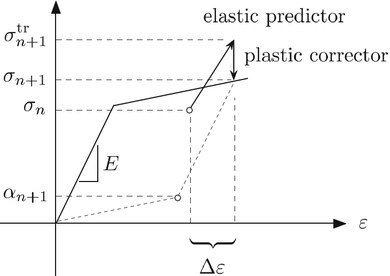Figure 4: Graphical representation of the return mapping algorithm .

For associative plasticity, during the plastic corrector step driven by the increment of the plasticity parameter ${\textstyle {\lambda }}$, the plastic flow direction ${\textstyle {r}}$ is normal to the yield surface.

For the ${\textstyle {J_{2}}}$ flow theory and associative plasticity, the return mapping is characterized to be ${\textstyle {radial}}$ . This because the von Mises yield surface is circular, thus its normal is also radial. In Figure 5 a graphical representation of the radial return algorithm for ${\textstyle {J_{2}}}$ plasticity is shown.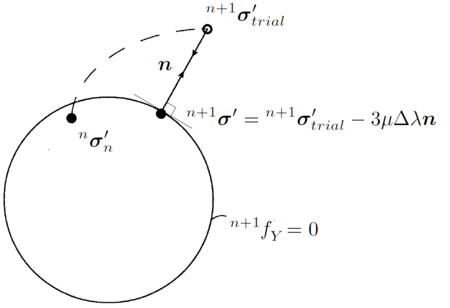Figure 5: Graphical representation of the radial return method for ${J_{2}}$ plasticity.

The return radial mapping procedure typically starts with the elastic prediction of the stresses. This means that the linear momentum equations are solved using only the elastic part of the continuum tangent modulus (Eq.(101)) and the stress tensor ${\textstyle {{\sigma }^{(0)}}}$ is computed with Eq.(114) (the superindex refers to the iteration index).

Then the effective stress ${\textstyle {\bar {\sigma }}}$ is computed with Eq.(121) and the yield function Eq.(119) is evaluated. If ${\textstyle {f_{Y}>0}}$ the return mapping iterative procedure is required. In fact, an elastoplastic step has been computed as purely elastic without fulfilling the consistency condition.

The first step of the iterative corrector procedure consists of computing the increment of the plastic parameter as

 $\delta \lambda _{pl}^{(k)}={\frac {f^{(k)}}{3\mu +H^{(k)}}}$
(140)

For perfect plasticity, the plastic modulus ${\textstyle {H}}$ is null, hence the plastic parameter is ${\textstyle {\delta \lambda _{pl}^{(k)}=f^{(k)}/(3\mu )}}$.

The plastic parameter increment is updated as

 $\Delta \lambda _{pl}^{(k+1)}=\Delta \lambda _{pl}^{(k)}+\delta \lambda _{pl}^{(k)}$
(141)

If before this step the material has never suffered plastic deformations, then ${\textstyle {\Delta \lambda _{pl}^{(k)}=0}}$.

Next the plastic strain and the internal variables are updated according to the plastic correction derived from Eq.(140).

The increment of plastic deformation is

 $\Delta \varepsilon _{pl}^{(k)}=-\delta \lambda _{pl}^{(k)}{\sqrt {\frac {3}{2}}}n$
(142)

So the total plastic deformations are

 $\varepsilon _{pl}^{(k+1)}=\varepsilon _{pl}^{(k)}+\Delta \varepsilon _{pl}^{(k)}$
(143)

Once again, for the first plastic step ${\textstyle {\varepsilon _{pl}^{(k)}=0}}$.

Next the deviatoric stresses are updated as

 $\sigma '^{(k+1)}=\sigma '^{(0)}-2\mu \Delta \lambda _{pl}^{(k+1)}r^{(0)}$
(144)

The plastic flow direction ${\textstyle {r}}$ remains unchanged because also the tensor ${\textstyle {n}}$ remains unchanged (and radial) during the plastic corrector phase (Eq.(138)). This is a particular feature of the radial return mapping.

From Eqs.(121,144), the updated effective stress is

 ${\bar {\sigma }}^{(k+1)}={\bar {\sigma }}^{(0)}-3\mu \Delta \lambda _{pl}^{(k+1)}$
(145)

Then the yield condition (Eq.119) is verified again with the updated effective stress. If it is not fulfilled, the steps from Eq.(140) to Eq.(144) are repited until ${\textstyle {f_{Y}^{(k)}=0}}$.

In Box 5, the return mapping algorithm for the ${\textstyle {J_{2}}}$ theory and referred to a general elastoplastic time interval ${\textstyle {t[^{n}t,{^{n+1}t}]}}$ is summarized.

### 2.3.4 Validation examples

In this section several problems are studied in order to validate and the V and the VP elements and to make interesting comparisons. First an example for small displacements is studied. Then a benchmark problem for non-linear solid mechanics, namely the Cook's membrane, is analyzed. The third example is a uniformly loaded circular plate and it involves also plasticity. All these examples are analyzed in statics considering a unique unit time step increment for the velocity-based formulations. The last example is solved in dynamics and for both the hypoelastic and hypoelastic-plastic models.

Simply supported beam

The first validated problem is a simply supported beam loaded by its self-weight. The problem has been studied in statics so the inertial forces have not been considered. The geometry of the problem is illustrated in Figure 6a and the problem data are given in Table 2.1.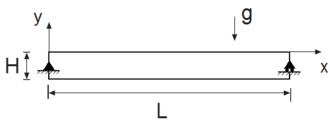Figure 6: Simply supported beam. Initial geometry.
Table 2.1. Simply supported beam. Problem data.
 L 5 m H 0.5 m Young modulus 196 GPa Density $7.85\times 10^{3}~kg/m^{3}$ Poisson ratio 0

The material properties of the structure can be assimilated to the ones of a structural steel.

The beam undergoes small displacements under the effect of its self-weight, hence linear elastic theory is suitable for computing a reference solution. The accuracy of the formulation is tested by comparing the computed values for the maximum vertical displacement and the maximum XX-component of the Cauchy stress tensor with the values given by a linear elastic analysis. According to this theory, both maximum values are reached in the central section of the beam and they are computed as

 $U_{Y}^{max}={\frac {5gHL^{4}}{384EI}}=1.5348\cdot {10}^{-4}m$
(146)

 ${\sigma }_{X}^{max}={\frac {3gHL^{2}}{4H^{2}}}=2887818Pa$
(147)

The problem has been solved in 2D using both the Velocity and the mixed Velocity-Pressure formulations using 3-noded triangular elements. The static problem is solved with only one unit time increment.

In order to verify the convergence of both schemes, the problem has been solved using structured meshes of 3-noded triangles with the following average sizes: 0.25m, 0.125m, 0.05m, 0.025m, 0.0125m. In Figure 7 the finest (mesh size=0.00125m, 32000 elements) and the coarsest (mesh size=0.25m, 80 elements) meshes are illustrated.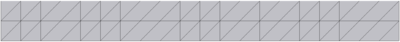(a) average mesh size= 0.25m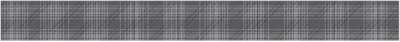(b) average mesh size= 0.0125m Figure 7: Simply supported beam. Coarsest and finest meshes used for the analysis.

In Figure 8 the solutions for the vertical displacement and the XX-component of the Cauchy stress tensor computed at the Gauss points obtained with the mesh with average size 0.025m are plotted.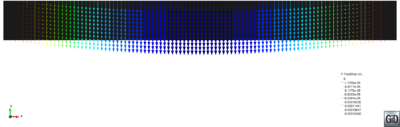(a) Displacements in Y-direction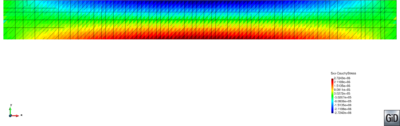(b) Cauchy stress, XX-component Figure 8: Simply supported beam. Numerical results.

For the visualization of all the numerical results of this work the pre-postprocessor software GID  has been used.

Table 2.2 collects the values of the maximum vertical displacement (absolute value) and the XX-component of the Cauchy stress tensor computed at the Gauss point using the V-element and the VP-element for different FEM mesh.

Table 2.2. Simply supported beam. Cauchy stress XX-component and maximum vertical displacement for different discretizations.
 mesh V-element VP-element size $\sigma _{x}^{max}$ $U_{y}^{max}$ $\sigma _{x}^{max}$ $U_{y}^{max}$ 2.50E-01 1.46E+06 9.92E-05 1.53E+06 8.41E-05 1.25E-01 2.29E+06 1.37E-04 2.37E+06 1.29E-04 5.00E-02 2.72E+06 1.54E-04 2.80E+06 1.52E-04 2.50E-02 2.82E+06 1.56E-04 2.87E+06 1.56E-04 1.25E-02 2.86E+06 1.57E-04 2.89E+06 1.57E-04

In the examples presented in this section, for the convergence analysis the percentage error is computed versus the solution obtained with the finest discretization as

 $error=abs\left({\frac {value_{finest~mesh}-value_{tested~mesh}}{value_{finest~mesh}}}\right)\cdot 100$
(148)

For example, the maximum vertical displacement obtained with the finest mesh (average size 0.0125m) is the reference solution for both V and VP elements. The convergence curves for both formulations are plotted in Figure 9. Both elements show a quadratic convergence rate for this error measure.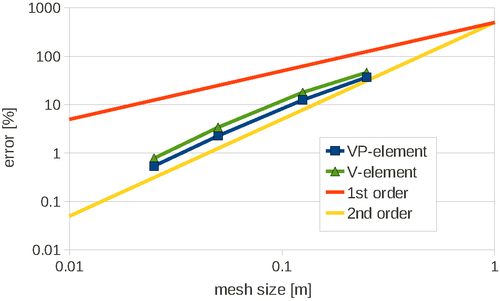Figure 9: Simply supported beam. Convergence analysis for the maximum vertical displacement for V and VP elements.

Compressible Cook's membrane

The Cook's membrane is a benchmark problem for solid mechanics. The static problem is solved twice in this thesis. In this section a compressible material is considered; in the next chapter the nearly incompressible case is analyzed. In both cases the problem has been solved with only one unit time increment.

The initial geometry of the problem, as well the problem data are given in Figure 10a.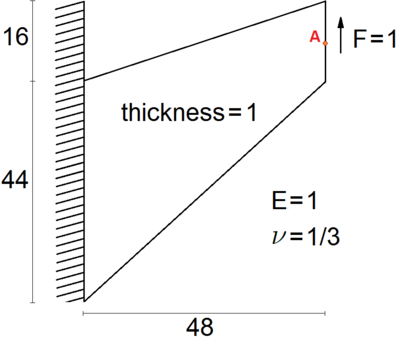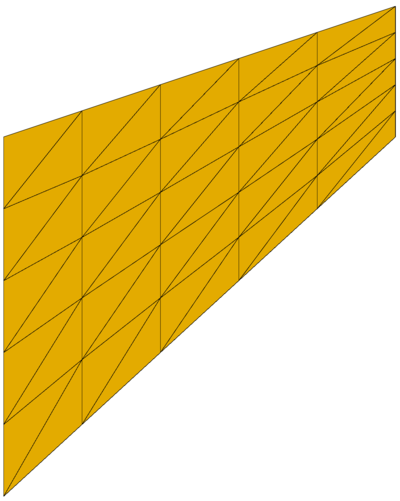(a) Initial geometry (b) FEM mesh Figure 10: Cook's membrane. Initial geometry, material data and FEM mesh (5 subdivisions for each edge corresponding to 50 elements).

The self weight of the membrane has not been taken into account in the analysis, so the membrane deforms under only the effect of the external load applied at its free edge. In this case the structure undergoes large displacements and the solution cannot been computed analytically. The results taken as the reference ones are those published in . The comparison with the mentioned publication, as well the convergence test are performed for the vertical displacement of point A of Figure 10a with coordinates ${\textstyle (x,y)=(48,52)}$. According to , under plane stress conditions this displacement is ${\textstyle U_{Y}^{max}=23.964}$.

The domain is discretized with a structured mesh and the edges of the membrane have the same number of partitions. In Figure 10 one of the meshes used for this problem is given. The figure refers to the case of 5 elements for each edge of the membrane.

For the 2D simulation a convergence study has been performed using various discretizations. In the finest one the edges have 200 subdivisions, while in the coarsest one only 2.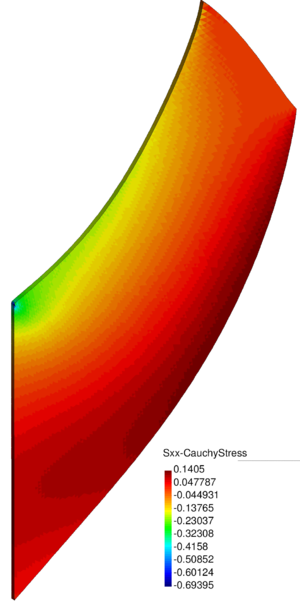Figure 11: Cook's membrane. Numerical results for the 3D simulation: the XX-component of the Cauchy stress tensor is plotted over the deformed configuration.

The 3D problem (thickness=1) has been solved for an unstructured mesh with average size 0.5 only. The results for this mesh are given in Figure 11, where the XX-component of the Cauchy stress tensor is plotted over the deformed configuration.

For the 3D simulation, the vertical displacements at the central point of the free edge for the V and the VP elements are 23.942 and 23.952, respectively, which correspond to an error versus the reference solution of 0.092% for the V-element and 0.050% for the VP-element.

The vertical displacement of point A of Figure 10a obtained for all the 2D discretizations and for both the Velocity and the Velocity-Pressure formulations is plotted in the graph of Figure 12.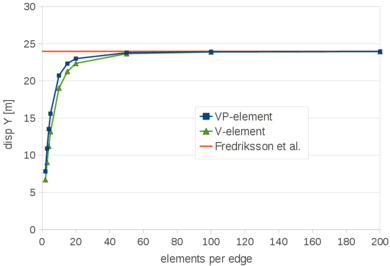Figure 12: Cook's membrane. Vertical displacement of point A of Figure 10a. Results for V and VP elements compared to the reference solution .

In Table 2.3 the numerical values are given.

Table 2.3. Cook's membrane. Maximum vertical displacement for different discretizations.

 elements V-element VP-element per edge $U_{y}^{max}$ $U_{y}^{max}$ 2 6.707 7.8105 3 9.0274 10.901 4 11.232 13.515 5 13.1755 15.5985 10 19.037 20.729 15 21.272 22.332 20 22.349 22.987 50 23.658 23.781 100 23.878 23.913 200 23.941 23.95

The convergence curves are given in Figure 13.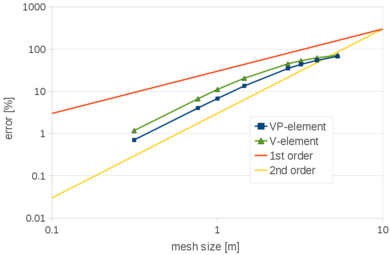Figure 13: Cook's membrane. Convergence analysis for the vertical displacement of point A of Figure 10a for the V and VP elements, V-element and VP-element, respectively.

Also in this case the convergence rate is quadratic for both formulations.

The problem analyzed in this section is a simply supported circular plate subjected to a uniform pressure ${\textstyle P}$ on its top surface. The plate constrains are applied on its lower edge. The plate has a radius ${\textstyle R=10}$ and thickness ${\textstyle h=1}$. In this work, the axial symmetry of the problem has not been used, and the plate has been analyzed in 3D using 4-noded tetrahedra. The average size for the tetrahedra is 0.175. This gives 214047 nodes. In Figure 14 the FEM mesh used is shown.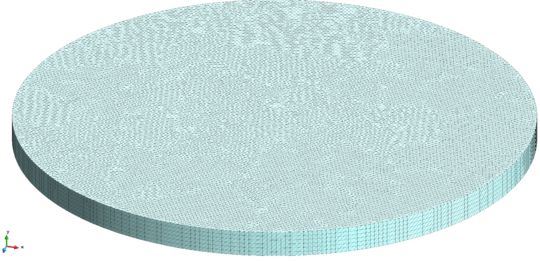Figure 14: Uniformly loaded circular plate. Initial geometry and 3D FEM used.

A hypoelastic-perfectly plastic model has been used, and the problem has been solved with the mixed velocity pressure formulation. For the plastic part, a von Mises yield criterion has been considered. The plate has Young modulus ${\textstyle E=10^{7}}$, Poisson ratio ${\textstyle \nu =0.24}$ and a uniaxial yield stress ${\textstyle {\bar {\sigma }}_{y}=16000}$. The objective of the study is to determine the limit load for the plate. Using the procedure described in , the limit load can be computed analytically by combining the limit analysis and the finite difference method. According to this theory, the limit load can be approximated as

 $P_{lim}\approx {\frac {1.63{\bar {\sigma }}_{y}h^{2}}{R^{2}}}=260.8$
(149)

The same problem was solved in  using eight-noded axisymmetric quadrilateral elements with four Gauss integration points. The limit load obtained with a relatively coarse mesh (10 finite elements distributed in two layers across the thickness) is ${\textstyle P_{lim}^{FE}=259.8}$ .

As in , the limit load has been considered as the one for which the non-linear procedure cannot longer converge for a small increment of the load.

In Figure 15 the maximum vertical displacement of the plate is plotted against the pressure on the top surface. In Table 2.4 the numerical values are given.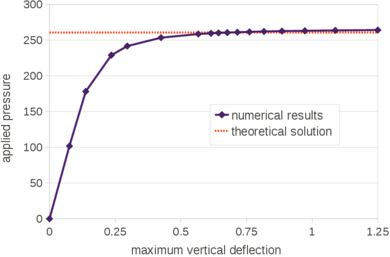Figure 15: Uniformly loaded circular plate. Maximum deflection versus the applied pressure.

Table 2.4.. Uniformly loaded circular plate. Numerical values of the maximum vertical deflection for different applied pressures.
 pressure max. deflection pressure max. deflection 101.84 0.0758 260.71 0.677 178.22 0.138 261.21 0.716 229.14 0.236 261.73 0.761 241.87 0.296 262.24 0.816 253.58 0.424 262.73 0.885 258.67 0.567 263.26 0.972 259.69 0.615 263.77 1.088 260.20 0.644 264.27 1.250

For the present analysis the limit load obtained is ${\textstyle P_{lim}=264.27}$, the relative percentage errors with respect the solutions given in  and  are 1.37% and 1.76%, respectively.

In Figure 16 the vertical displacements are depicted over the deformed configuration obtained with the limit load. The plate central section is highlighted in the picture.

In Figures 17 and 18 some snapshots of the von Mises effective stress are plotted over the central section of the plate for the different load conditions. The picture shows clearly the progressive evolution of the plastic zone.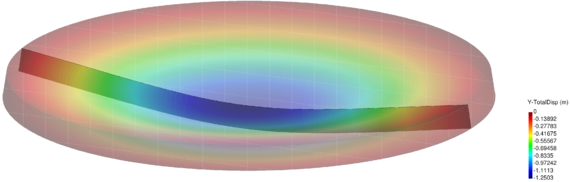Figure 16: Uniformly loaded circular plate. Vertical displacement contours for the maximum pressure sustained by the plate (${P_{lim}=264.27}$).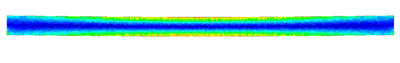(a) Overall load=101.84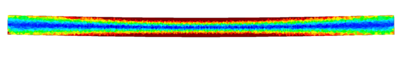(b) Overall load=178.22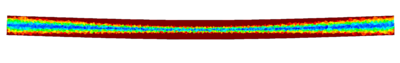(c) Overall load=229.14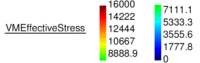Figure 17: Uniformly loaded circular plate. Von Mises effective stress over the deformed configurations for different load conditions (only the central section is depicted) (I/II).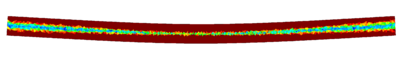(a) Overall load=253.58(b) Overall load=261.73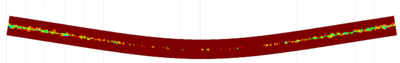(c) Overall load=264.27Figure 18: Uniformly loaded circular plate. Von Mises effective stress over the deformed configurations for different load conditions (only the central section is depicted) (II/II).

Plane strain cantilever in dynamics

The plane strain cantilever illustrated in Figure 19a has been chosen as the reference case for a large displacement dynamics analysis. The problem data are in given in Table 2.5. The problem was introduced and studied in . In the reference publication, the load was applied as a step function at time ${\textstyle t=0s}$ and its magnitude was defined by the equation ${\textstyle f=15(1-y^{2}/4)}$ where ${\textstyle {y}}$ is the coordinate in the direction of the load. However, in this analysis the load has been considered uniformly distributed over the free edge (the overall value is 40, as in ).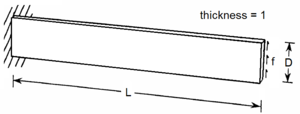Figure 19: Plane strain cantilever. Initial geometry.
Table 2.5. Plane strain cantilever. Problema data.
 L 25 D 4 Young modulus $10^{4}$ Poisson ratio 0.25

The problem has been solved with both a hypoelastic and a hypoelastic-plastic models. First the results of the hypoelastic model are given.

Hypoelastic model

The problem has been solved in 2D and 3D and using both the V and VP elements. In order to simulate the plane strain state, in the 3D analysis the nodal displacements in the transversal direction to the load have been constrained .

The reference solution is the elastic one given in .

For the 2D analysis a convergence study has been performed. Structured finite element meshes have been used and the coarsest and the finest ones have a mean size of 1 and 0.125, respectively. Both meshes are given in Figure 20.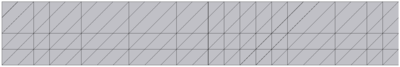(a) Average mesh size= 1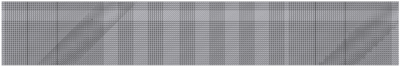(b) Average mesh size= 0.125 Figure 20: Plane strain cantilever. Coarsest (mesh size=1, 200 elements) and finest meshes (mesh size=0.125, 12800 triangles) used for the 2D analysis.

For the 3D case, the problem has been solved with the finest mesh only (average size for the 4-noded tetrahedra equal to 0.125). The results for the 3D case obtained with the VP-element are illustrated in Figure 21 where the pressure contours are plotted over the deformed configuration.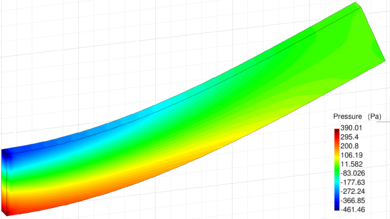Figure 21: Plane strain cantilever. Numerical results for the 3D simulation obtained with the VP-element: pressure contours plotted over the deformed configuration.
In Figure 22 the time evolution of the top corner vertical displacement is plotted for each of the FEM meshes. These results have been obtained with the VP-element.Figure 22: Plane strain cantilever. Time evolution of the top corner vertical displacement for different 2D discretizations. Results obtained with the VP-element.

According to , the maximum vertical displacement is ${\textstyle U_{Y}^{max}=6.88}$. Table 2.6 collects the maximum vertical displacement obtained with the V and the VP elements for all the meshes.

Table 2.6. Plane strain cantilever. Maximum top corner vertical displacement for different 2D discretizations.
 mesh V-element VP-element size $U_{y}^{max}$ $U_{y}^{max}$ 1 5.759 6.306 0.8 6.144 6.534 0.5 6.568 6.743 0.25 6.811 6.863 0.125 6.875 6.895

The four curves of Figure 23 are the converged time evolution of the top corner vertical displacement obtained with the V-element in 3D, the VP-element in 2D and 3D and the reference solution . The curves corresponding to the V and VP elements are almost superposed and they match the reference solution.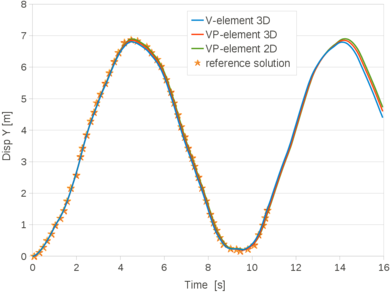Figure 23: Plane strain cantilever. Time evolution of the top corner vertical displacement. Solutions for the 2D VP-element and the 3D V and VP elements obtained with the finest mesh (average size 0.125) compared to the reference solution .

Hypoelastic-plastic model

The same problem has been solved for an elastic-plastic material with linear hardening. The yield stress is 300 and the plastic modulus ${\textstyle H}$ is 100. The problem has been solved with the mixed velocity-pressure formulation and by using structured meshes, as the ones of Figure 20. The reference solution is taken from  where the benchmark was proposed. In  the converged value for the maximum top corner vertical displacement is 8.22. The hypoelastic-plastic mixed velocity-pressure formulation converges to 7.97 (error of 2.998% In the graph of Figure 24 the time evolution of the top corner vertical displacement is plotted for the different FEM meshes.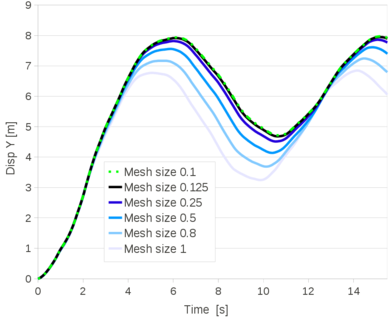Figure 24: Plane strain elastoplastic cantilever. Time evolution of the top corner vertical displacement for different 2D discretizations.

In Table 2.7 the numerical values for the maximum and the residual top corner vertical displacements are given for each of the FEM mesh.

Table 2.7. Plane strain elastoplastic cantilever. Maximum and residual top corner vertical displacements for different discretizations.
 mesh size $U_{y}^{max}$ $U_{y}^{res}$ 1 6.77 3.25 0.8 7.17 3.69 0.5 7.56 4.14 0.25 7.82 4.51 0.125 7.92 4.68 0.1 7.94 4.72 0.0625 7.97 4.77

The problem has been solved also for the 3D problem for a structured mesh of 4-noded tetrahedra with average size 0.125.

In Figures 25 the von Mises effective stresses are plotted over the deformed configuration at the time instant when the top corner vertical displacement is reached (${\textstyle t=6.05s}$).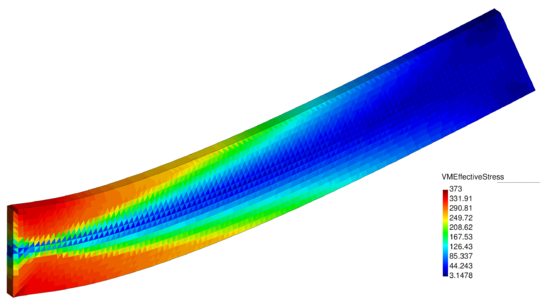Figure 25: Plane strain elastoplastic cantilever. Numerical results for the 3D simulation. Von Mises effective stress plotted over the deformed configuration at ($t=6.05s$).

In Figure 26 for the same time instant the XX-component of the Cauchy stress tensor is plotted.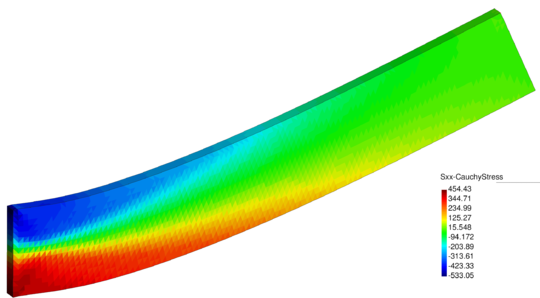Figure 26: Plane strain elastoplastic cantilever. Numerical results for the 3D simulation: XX-component of the Cauchy stress tensor plotted over the deformed configuration at ($t=6.05s$).

In Figure 27 the 3D solution is compared to the 2D results obtained with a structured mesh with the same average size. The curves coincide almost exactly.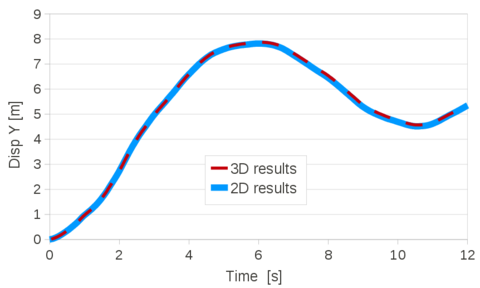Figure 27: Plane strain elastoplastic cantilever. Time evolution of the top corner vertical displacement. Numerical results for the 2D and the 3D simulations for the same average mesh size (0.125).

## 2.4 Summary and conclusions

In this chapter two velocity-based finite element Lagrangian procedures, namely the Velocity and the mixed Velocity-Pressure formulations, have been derived for a general compressible material.

The derivation of both formulations has been carried out with the aim of maintaining the scheme as general as possible. The mixed Velocity-Pressure formulation has been derived exploiting the linearized form of the Velocity formulation. In particular, it has been shown that the tangent matrix of the linear momentum equations is the same for both schemes.

The mixed Velocity-Pressure procedure is based on a two-step Gauss-Seidel solution algorithm. First, the linear momentum equations are solved for the velocity increments, next the continuity equation is solved for the pressure in the updated configuration. At the end of these steps the convergence for the velocities and the pressure is checked. Linear interpolation has been used for both velocity and pressure fields.

Next, both formulations have been particularized for hypoelastic solids. The finite elements generated from the Velocity formulation and the mixed Velocity-Pressure formulations have been called V and VP element, respectively.

The numerical scheme for dealing with ${\textstyle J_{2}}$ associative plasticity has been also given.

Several numerical examples have been given for validating the V and VP elements for large displacements dynamics problems involving both hypoelastic and hypoelastoplastic compressible solids. It has been shown that both elements are convergent for all the numerical examples analyzed.

# Chapter 3. Unified stabilized formulation for quasi-incompressible materials

This chapter is devoted to the derivation and validation of the unified stabilized formulation for nearly-incompressible materials. Namely, the cases of quasi-incompressible Newtonian fluids and the quasi-incompressible hypoelastic solids will be analyzed.

Quasi-incompressible materials have a compressibility that is small enough to neglect the variation of density on time but, unlike fully incompressible materials, they are not totally divergence-free and the volumetric strain rate is related to the variation on time of pressure via Eq.(75). This stabilized formulation is based on the mixed velocity-pressure formulation derived in the previous chapter for a general material. In fact a one-field method, as the Velocity formulation presented in Chapter 2, is not sufficient for dealing with the incompressibility constraint. Furthermore the ${\textstyle inf-sup}$ condition  imposes the stabilization of the mixed finite element procedure, if an equal order interpolation is used for the velocities and the pressure, as in this work. Consequently, the mixed Velocity-Pressure formulation derived for compressible materials in the previous chapter needs to be stabilized in order to solve quasi-incompressible problems.

In this work a new stabilized Lagrangian method for quasi-incompressible materials is derived. The stabilization procedure is based on the consistent derivation of a residual-based stabilized expression of the mass balance equation using the ${\textstyle {FiniteCalculus(FIC)}}$, also called ${\textstyle {FiniteIncrementCalculus}}$ method [ [43,76,92,94,96–98]. The main ideas of this part of the thesis are taken from  where the stabilization technique was derived for homogeneous viscous fluids. In this chapter it is shown that the procedure can be extended also for the analysis of quasi-incompressible solids.

The FIC approach in mechanics is based on expressing the equations of balance of mass and momentum in a space-time domain of finite size and retaining higher order terms in the Taylor series expansion typically used for expressing the change in the transported variables within the balance domain. In addition to the standard terms of infinitesimal theory, the FIC form of the balance equations contains derivatives of the classical differential equations in mechanics multiplied by characteristic distances in space and time.

In this work the second order FIC form in space and the first order FIC form in time of the mass balance equation have been used as the basis for the derivation of the stabilized formulation. The discretized variational form of the FIC mass balance equation via the FEM introduces terms in the Neumann boundary of the domain and other terms involving the first and second material time derivatives of the pressure. These terms are relevant for ensuring the consistency of the residual formulation.

The FIC stabilization, although is derived using the linear momentum equations, affects only the continuity equation. This means that the general form of the discretized and linearized momentum equations derived in Chapter 2 for the mixed Velocity-Pressure formulation still holds for quasi-incompressible materials. Hence, for hypoelastic quasi-incompressible materials the linear momentum equations are solved through the same linear system derived for the VP (compressible) element in Section 2.3.2. To avoid repetitions, the linearized momentum equations for the mixed velocity-pressure formulation will be recalled only for Newtonian fluids by deriving the tangent matrix and the internal forces according to the constitutive law.

For convenience, the stabilized form of the continuity equation is derived for quasi-incompressible Newtonian fluids, as in . Nevertheless it will be shown that the approach can be easily extended to quasi-incompressible hypoelastic solids.

For the fluid analysis a Lagrangian procedure called Particle Finite Element Mehtod (PFEM) [88+ is used. With the PFEM, the mesh nodes are treated as particles and they move according to the governing equations. The domain is continuously remeshed using a procedure that efficiently combines the Delaunay tessellation and the Alpha Shape Method .

The FIC stabilized formulation here presented  has excellent mass preservation feature in the analysis of free surface fluid problems. Preservation of mass is a great challenge in the numerical study of flow problems with high values of the bulk modulus that approach the conditions of incompressibility. Mass losses can be induced by the stabilization terms which are typically added to the discretized form of the momentum and mass balance equations in order to account for high convective effects in the Eulerian description of the flow, and to satisfy the ${\textstyle inf-sup}$ condition imposed by the full incompressibility constraint when equal order interpolation of the velocities and the pressure is used in mixed FEMs [9,38,135,137].

An important source of mass loss emanates in the numerical solution of free surface flows due, among other reasons, to the inaccuracies in predicting the shape of the free surface during large flow motions . Mass losses can also occur in the numerical solution of flows with heterogeneous material properties  and in homogeneous viscous flows using the Laplace form of the Navier-Stokes equations .

In Lagrangian analysis procedures (such as the PFEM) the motion of the fluid particles is tracked during the transient solution. Hence, the convective terms vanish in the momentum equations and no numerical stabilization is needed for treating those terms. Two other sources of mass loss, however, remain in the numerical solution of Lagrangian flows, i.e. that due to the treatment of the incompressibility constraint by a stabilized numerical method, and that induced by the inaccuracies in tracking the flow particles and, in particular, the free surface.

The discretized variational form of the FIC mass balance equation via the FEM introduces terms in the Neumann boundary of the domain, and other terms involving the first and second material time derivatives of the pressure that are relevant for ensuring the consistency of the residual formulation. These terms are also crucial for preserving the mass during the transient solution of free surface Lagrangian flows. In addition they enable the computation of the nodal pressures from the stabilized mass balance equation without imposing any condition on the pressure at the free surface nodes, thus eliminating another source of mass loss which occurs when the pressure is prescribed to a zero value on the free surface in viscous flows.

A section of this chapter is exclusively dedicated to show the excellent mass preservation features of the PFEM-FIC stabilized formulation for free surface flow problems.

Various approaches have been developed in the recent years for approximating fluid flows by means of a quasi-incompressible material. In practice, they all consider the Navier-Stokes problem with a modified mass conservation equation where a slight compressibility is added to the fluid. In previous works, see for example [27,92,122,137], the fluid compressibility was introduced by relaxing the incompressibility constraint by means of a penalty parameter. Alternatively, the small compressibility of the fluid can be introduced by considering the actual bulk modulus of the fluid ${\textstyle {\kappa }}$, which gives this operation a physical meaning, [38,63,137]. The success of quasi-incompressible formulations in fluid mechanics relies on their important advantages from the numerical point of view. The most obvious one is that the quasi-incompressible form of the continuity equation yields a direct relation between the two unknown fields of the Navier-Stokes problem, the velocities and the pressure (Eq.(75)). This is useful if the problem is solved using a partitioned scheme because the velocity-pressure relation is crucial for deriving the tangent matrix of the momentum equations. Furthermore, another important drawback of fully incompressible schemes is eluded; the incompressibility constraint leads to a diagonal block of a zero matrix in the global matrix system. Consequently, a pivoting procedure is required to solve numerically this kind of linear system. It is well known that the computational cost associated to this operation is high and it increases with the number of degrees of freedom of the problem. The compressibility terms that emanate from the quasi-incompressible form of the continuity equation fill the diagonal of the global matrix, thus overcoming these numerical difficulties.

On the other hand, quasi-incompressible schemes insert in the numerical model parameters that have typically high values and can lead to different numerical instabilities. For example, large values of the penalty parameter or, equally, physical values of the bulk modulus, can compromise the quality of the analyses, or even prevent the convergence of the solution scheme, . For this reason, generally, the value of the actual bulk modulus is reduced arbitrarily to the so-called ${\textstyle {pseudobulkmodulus}}$. Nevertheless, an excessively small value of the pseudo bulk modulus changes drastically the meaning of the continuity equation of the original Navier-Stokes problem. In other words, the incompressibility constraint would not be satisfied at all. Furthermore, the bulk modulus is proportional to the speed of sound propagating through the material. Hence, we have to guarantee that the order of magnitude of the velocities of the problem is several times smaller than the velocity of sound in the medium.

In this work, the pseudo-bulk modulus ${\textstyle \kappa _{p}}$ is used for the tangent matrix of the linear momentum equations, while the actual physical value of the bulk modulus ${\textstyle \kappa }$ is used for the numerical solution of the mass conservation equation. The pseudo bulk modulus is computed as a proportion of the real bulk modulus of the fluid through the parameter ${\textstyle \theta }$ such that ${\textstyle \kappa _{p}=\theta \kappa }$ with ${\textstyle 0<\theta \leq 1}$. A new numerical strategy for computing a priori the optimum value for the pseudo bulk modulus is also derived. For free surface flow problems, it will be shown that the scheme guarantees the good conditioning of the linear system, good convergence and excellent mass preservation features.

The lay-out of this chapter is the following. First the stabilized FIC form of the mass balance equation is derived. The procedure is described for a Newtonian fluid from the local and continuous form until the fully discretized matrix form. Next the linearized and discretized expression of the linear momentum equations derived in Chapter 2 for the mixed Velocity-Pressure formulation is adapted for Newtonian fluids and the complete solution scheme is given. Next the FIC stabilization procedure is extended to hypoelastic quasi-incompressible materials and the complete solution scheme is given also for this constitutive model.

In Section 3.4, free surface flows are analyzed in detail. First the essential features of the PFEM are given, then the mass preservation feature of the PFEM-FIC stabilized formulation is shown together with some explicative numerical examples. Finally the conditioning of the linear system is studied and a practical and efficient technique to predict ${\textstyle apriori}$ the optimum value for the pseudo bulk modulus is given.

The chapter ends up with several validation examples for quasi-incompressible solid and fluid mechanics problems.

## 3.1 Stabilized FIC form of the mass balance equation

The FIC stabilized form of the continuity equation is here derived. For convenience, the derivation procedure is carried out for quasi-incompressible Newtonian fluids.

### 3.1.1 Governing equations

For the sake of clarity, the local forms of the linear momentum ${\textstyle {r_{m}}_{i}}$ and the continuity ${\textstyle r_{v}}$ equations are recalled. From Eqs.(1) and (75) yields

 ${r_{m}}_{i}:=\rho {\partial v_{i} \over \partial t}-{\partial \sigma _{ij} \over \partial x_{j}}-b_{i}=0\quad ,\quad i,j=1,n_{s}\quad {\hbox{in }}\Omega$
(150)

 $r_{v}:=-{\frac {1}{\kappa }}{\partial p \over \partial t}+d^{v}=0$
(151)

The terms of Eqs.(150 and 151) have already been defined in the previous chapters.

The standard form of the constitutive relation for a Newtonian fluid reads

 $\sigma _{ij}=\sigma _{ij}'+p\delta _{ij}=2\mu d_{ij}'+p\delta _{ij}$
(152)

where ${\textstyle \mu }$ is the viscosity and the deviatoric strain rate ${\textstyle d_{ij}'}$ is defined from Eq.(71) as

 $d_{ij}'=d_{ij}-{1 \over 3}d^{v}\delta _{ij}$
(153)

where ${\textstyle d^{v}}$ is the volumetric strain rate.

Substituting Eqs.(152) and (153) into (150), gives a useful form of the momentum equations for the Newtonian fluids as

 $\rho {\partial v_{i} \over \partial t}-{\partial \over \partial x_{j}}(2\mu d_{ij})+{\partial \over \partial x_{i}}\left({\frac {2}{3}}\mu d^{v}\right)-{\partial p \over \partial x_{i}}-b_{i}=0\quad ,\quad i,j=1,n_{s}$
(154)

### 3.1.2 FIC mass balance equation in space and in time

Previous stabilized FEM formulations for quasi and fully incompressible fluids and solids were based on the first order form of the Finite Calculus (FIC) balance equation in space [76-85,92,97,98]. In this work, for the derivation of stabilized formulation both the second order FIC form of the mass balance equation in space [92,96] and the first order FIC form of the mass balance equation in time are used. These forms read respectively I

 $r_{v}+{\frac {h_{i}^{2}}{12}}{\frac {\partial ^{2}r_{v}}{\partial x_{i}^{2}}}=0\qquad {\hbox{in }}\Omega \qquad i=1,n_{s}$
(155)

and

 $r_{v}+{\frac {\delta }{2}}{\partial r_{v} \over \partial t}=0\qquad {\hbox{in }}\Omega$
(156)

Eq.(155) is obtained by expressing the balance of mass in a rectangular domain of finite size with dimensions ${\textstyle h_{1}\times h_{2}}$ (for 2D problems), where ${\textstyle h_{i}}$ are arbitrary distances, and retaining up to third order terms in the Taylor series expansions used for expressing the change of mass within the balance domain. The derivation of Eq.(155) for 2D incompressible flows can be found in .

Eq.(156), on the other hand, is obtained by expressing the balance of mass in a space-time domain of infinitesimal length in space and finite dimension ${\textstyle \delta }$ in time .

The FIC terms in Eqs.(155) and (156) play the role of space and time stabilization terms respectively. In the discretized problem, the space dimensions ${\textstyle h_{i}}$ and the time dimension ${\textstyle \delta }$ are related to characteristic element dimensions and the time step increment, respectively as it will be explained later.

Note that for ${\textstyle h_{i}\to 0}$ and ${\textstyle \delta \to 0}$ the standard form of the mass balance equation (151), as given by the infinitesimal theory, is recovered.

### 3.1.3 FIC stabilized local form of the mass balance equation

Substituting Eq.(151) into Eqs.(155) and (156) the second order FIC form in space and the first order FIC form in time of the mass balance equation for a general quasi-incompressible material read

 $-{\frac {1}{\kappa }}{\partial p \over \partial t}+d^{v}-{\frac {h_{i}^{2}}{12}}{\frac {\partial ^{2}}{\partial x_{i}^{2}}}\left({\frac {1}{\kappa }}{\partial p \over \partial t}\right)+{\frac {h_{i}^{2}}{12}}{\frac {\partial }{\partial x_{i}}}\left({\frac {\partial d^{v}}{\partial x_{i}}}\right)=0\qquad {\hbox{in }}\Omega \qquad i=1,n_{s}$
(157)

and

 $-{\frac {1}{\kappa }}{\partial p \over \partial t}+d^{v}-{\frac {\delta }{2\kappa }}{\partial ^{2}p \over \partial t^{2}}+{\frac {\delta }{2}}{\partial d^{v} \over \partial t}=0$
(158)

The FIC form of the mass balance equation is expressed in terms of the momentum equations. Neglecting the space changes of the viscosity ${\textstyle \mu }$, from Eq.(154) the following expression is obtained

 ${\frac {2}{3}}\mu {\frac {\partial d^{v}}{\partial x_{i}}}=-\rho {\partial v_{i} \over \partial t}+2\mu {\partial \over \partial x_{j}}(d_{ij})+{\partial p \over \partial x_{i}}+b_{i}=-\rho {\partial v_{i} \over \partial t}+{\hat {r}}_{m_{i}}$
(159)

Hence

 ${\frac {\partial d^{v}}{\partial x_{i}}}={\frac {3}{2\mu }}\left[-\rho {\partial v_{i} \over \partial t}+{\hat {r}}_{m_{i}}\right]$
(160)

In the above two equations ${\textstyle {\hat {r}}_{m_{i}}}$ is a static momentum term defined as

 ${\hat {r}}_{m_{i}}=2\mu {\partial \over \partial x_{j}}(d_{ij})+{\frac {\partial p}{\partial x_{i}}}+b_{i}$
(161)

Substituting Eq.(160) into Eq.(157) and neglecting the space changes of ${\textstyle c}$ and ${\textstyle \rho }$ in the derivatives, the following form is obtained

 $-{\frac {1}{\kappa }}{\partial p \over \partial t}+d^{v}-{\frac {h_{i}^{2}}{12}}{\frac {\partial ^{2}}{\partial x_{i}^{2}}}\left({\frac {1}{\kappa }}{\partial p \over \partial t}\right)+{\frac {h_{i}^{2}}{8\mu }}{\frac {\partial }{\partial x_{i}}}\left(-\rho {\partial v_{i} \over \partial t}+{\hat {r}}_{m_{i}}\right)=0$
(162)

Observation of the term involving the material derivative of ${\textstyle v_{i}}$ in Eq.(162) gives

 ${\frac {\partial }{\partial x_{i}}}\left(-\rho {\partial v_{i} \over \partial t}\right)=-\rho {\partial \over \partial t}\left({\partial v_{i} \over \partial x_{i}}\right)=-\rho {\partial d^{v} \over \partial t}$
(163)

Substituting Eq.(163) into (162) gives

 $-{\frac {1}{\kappa }}{\partial p \over \partial t}+d^{v}-{\frac {h_{i}^{2}}{12\kappa }}{\frac {\partial ^{2}}{\partial x_{i}^{2}}}\left({\partial p \over \partial t}\right)+{\frac {h_{i}^{2}}{8\mu }}\left(-\rho {\partial d^{v} \over \partial t}+{\partial {\hat {r}}_{m_{i}} \over \partial x_{i}}\right)=0$
(164)

From Eq.(158),

 $-{\partial d^{v} \over \partial t}=-{\frac {2}{\delta \kappa }}{\partial p \over \partial t}+{\frac {2}{\delta }}d^{v}-{\frac {1}{\kappa }}{\partial ^{2}p \over \partial t^{2}}$
(165)

Substituting Eq.(165) into Eq.(164) gives

 $-{\frac {1}{\kappa }}{\partial p \over \partial t}+d^{v}-{\frac {h_{i}^{2}}{12\kappa }}{\frac {\partial ^{2}}{\partial x_{i}^{2}}}\left({\partial p \over \partial t}\right)+{\frac {h_{i}^{2}}{8\mu }}\left(-{\frac {2\rho }{\delta \kappa }}{\partial p \over \partial t}+{\frac {2\rho }{\delta }}d^{v}-{\frac {\rho }{\kappa }}{\partial ^{2}p \over \partial t^{2}}+{\partial {\hat {r}}_{m_{i}} \over \partial x_{i}}\right)=0$
(166)

Multiplying Eq.(166) by ${\textstyle {\frac {8\mu }{h^{2}}}}$ gives, after grouping some terms,

 $-{\frac {1}{\kappa }}{\partial p \over \partial t}\left({\frac {8\mu }{h_{i}^{2}}}+{\frac {2\rho }{\delta }}\right)+d^{v}\left({\frac {8\mu }{h_{i}^{2}}}+{\frac {2\rho }{\delta }}\right)-{\frac {2\mu }{3\kappa }}{\frac {\partial ^{2}}{\partial x_{i}^{2}}}\left({\partial p \over \partial t}\right)-{\frac {\rho }{\kappa }}{\partial ^{2}p \over \partial t^{2}}+{\partial {\hat {r}}_{m_{i}} \over \partial x_{i}}=0$
(167)

After some further transformations,

 $-{\frac {1}{\kappa }}{\partial p \over \partial t}+d^{v}-{\frac {2\mu \tau }{3\kappa }}{\partial \over \partial x_{i}}\left({\partial \over \partial x_{i}}\left({\partial p \over \partial t}\right)\right)-\tau {\frac {\rho }{\kappa }}{\partial ^{2}p \over \partial t^{2}}+\tau {\partial {\hat {r}}_{m_{i}} \over \partial x_{i}}=0$
(168)

Where ${\textstyle \tau }$ is a stabilization parameter given by

 $\tau =\left({\frac {8\mu }{h^{2}}}+{\frac {2\rho }{\delta }}\right)^{-1}$
(169)

For transient problems the stabilization parameter ${\textstyle \tau }$ is computed as

 $\tau =\left({\frac {8\mu }{(l^{e})^{2}}}+{\frac {2\rho }{\Delta t}}\right)^{-1}$
(170)

where ${\textstyle \Delta t}$ is the time step used for the transient solution and ${\textstyle l^{e}}$ is a characteristic element length.

The coefficient ${\textstyle {\frac {2\mu \tau }{3\kappa }}}$ multiplying the second space derivatives of ${\textstyle {\partial p \over \partial t}}$ in Eq.(168) is much smaller than the coefficients multiplying the rest of the terms in this equation. Numerical tests have shown that the results are not affected by this term. Consequently, this second space derivative term will be neglected in the rest of this work.

Hence the FIC stabilized form of the mass balance equation is written as

 $-{\frac {1}{\kappa }}{\partial p \over \partial t}+d^{v}-\tau {\frac {\rho }{\kappa }}{\partial ^{2}p \over \partial t^{2}}+\tau {\partial {\hat {r}}_{m_{i}} \over \partial x_{i}}=0$
(171)

Note that the term ${\textstyle {\partial \over \partial x_{i}}\left(2\mu {\partial \over \partial x_{j}}(d_{ij})\right)}$ within ${\textstyle {\hat {r}}_{m_{i}}}$ in Eq.(171) (see the definition of ${\textstyle {\hat {r}}_{m_{i}}}$ in Eq.(161)) vanishes for a linear approximation of the velocity field. This is the case for the simplicial elements used in this work.

### 3.1.4 Variational form

Multiplying Eq.(171) by arbitrary (continuous) test functions ${\textstyle q}$ (with dimensions of pressure) and integrating over the analysis domain ${\textstyle \Omega }$ gives

 $\int _{\Omega }-{\frac {q}{\kappa }}{\partial p \over \partial t}d\Omega -\int _{\Omega }q{\frac {\rho }{\kappa }}{\partial ^{2}p \over \partial t^{2}}d\Omega +\int _{\Omega }qd^{v}d\Omega +\int _{\Omega }q\tau {\partial {\hat {r}}_{m_{i}} \over \partial x_{i}}d\Omega =0$
(172)

Integrating by parts the last integral in Eq.(172) (and neglecting the space changes of ${\textstyle \tau }$) yields

 $\int _{\Omega }\!-{\frac {q}{\kappa }}{\partial p \over \partial t}d\Omega -\!\int _{\Omega }q\tau {\frac {\rho }{\kappa }}{\partial ^{2}p \over \partial t^{2}}d\Omega +\!\int _{\Omega }qd^{v}d\Omega -\!\int _{\Omega }\tau {\partial q \over \partial x_{i}}{\hat {r}}_{m_{i}}d\Omega +\!\underbrace {\int _{\Gamma }q\tau {\hat {r}}_{m_{i}}n_{i}d\Gamma } _{BT}=0$
(173)

where ${\textstyle n_{i}}$ are the components of the unit normal vector to the external boundary ${\textstyle \Gamma }$ of ${\textstyle \Omega }$.

Using Eq.(160) an equivalent form for the boundary term BT of Eq.(173) is obtained as

 $BT=\int _{\Gamma }q\tau {\hat {r}}_{m_{i}}n_{i}d\Gamma =\int _{\Gamma }q\tau \left(\rho {\partial v_{i} \over \partial t}+{\frac {2\mu }{3}}{\partial d^{v} \over \partial x_{i}}\right)n_{i}d\Gamma =\int _{\Gamma }q\tau \left(\rho {\partial v_{n} \over \partial t}+{\frac {2\mu }{3}}{\partial d^{v} \over \partial n}\right)d\Gamma$
(174)

where ${\textstyle {\partial d^{v} \over \partial n}}$ is the derivative of the volumetric strain rate in the direction of the normal to the external boundary and ${\textstyle v_{n}}$ is the velocity normal to the boundary.

The term ${\textstyle {\frac {2\mu }{3}}{\partial d^{v} \over \partial n}}$ can be approximated as follows

 ${\frac {2\mu }{3}}{\partial d^{v} \over \partial n}={\frac {2}{h_{n}}}\left({\frac {2}{3}}\mu ^{+}d^{v+}-{\frac {2}{3}}\mu ^{-}d^{v-}\right)\quad {\hbox{at }}\Gamma$
(175)

where ${\textstyle (\mu ^{+},d^{v+})}$ and ${\textstyle (\mu ^{-},d^{v-})}$ are respectively the values of ${\textstyle \mu }$ and ${\textstyle d^{v}}$ at exterior and interior points of the boundary ${\textstyle \Gamma }$ and ${\textstyle h_{n}}$ is a characteristic length in the normal direction to the boundary. Figure 28 shows an example of the computation of ${\textstyle {\frac {2}{3}}\mu {\partial d^{v} \over \partial n}}$ at the side of a 3-noded triangle adjacent to the external boundary. The same procedure applies for 4-noded tetrahedra.